# R S Aggarwal Solutions for Class 11 Maths Chapter 13- Some Special Series

The R S Aggarwal Solutions for the questions given in Chapter 13, Some Special Series, of the Class 11 R S Aggarwal textbooks are available here. In this chapter, students learn about some special series, other than Arithmetic Progression and Geometric Progression, in detail. Students can easily score full marks in the exam by solving all the questions present in the R S Aggarwal textbook.

The Class 11 R S Aggarwal Solutions for Some Special Series are very easy to understand. These solutions cover all the exercise questions included in the book. Here, the PDF of the Class 11 maths Chapter 13 R S Aggarwal Solutions is available, which can be referred in online mode. Students can also avail these R S Aggarwal solutions and download it for free to practice them offline as well.

### Download PDF of R S Aggarwal Solutions for Class 11 Maths Chapter 13- Some Special Series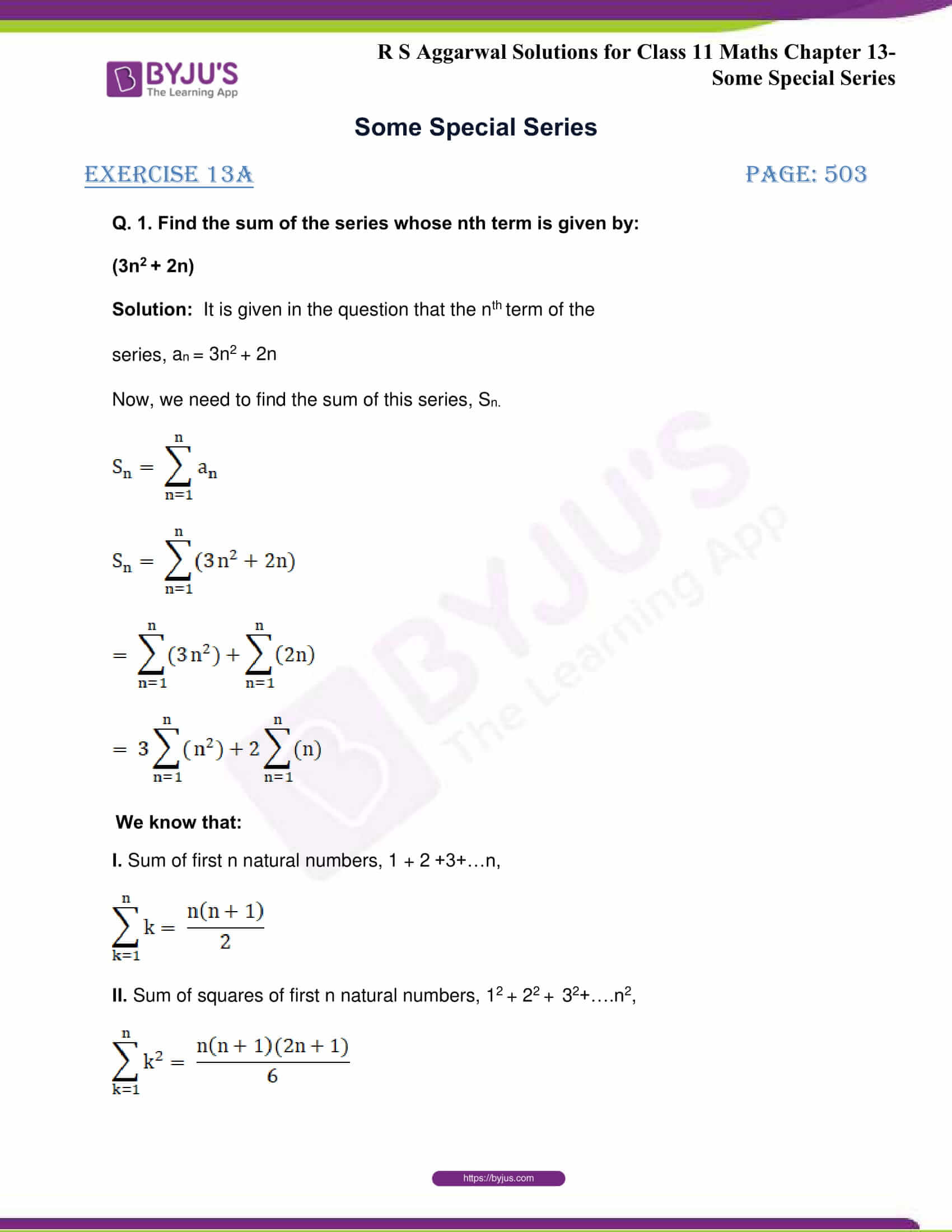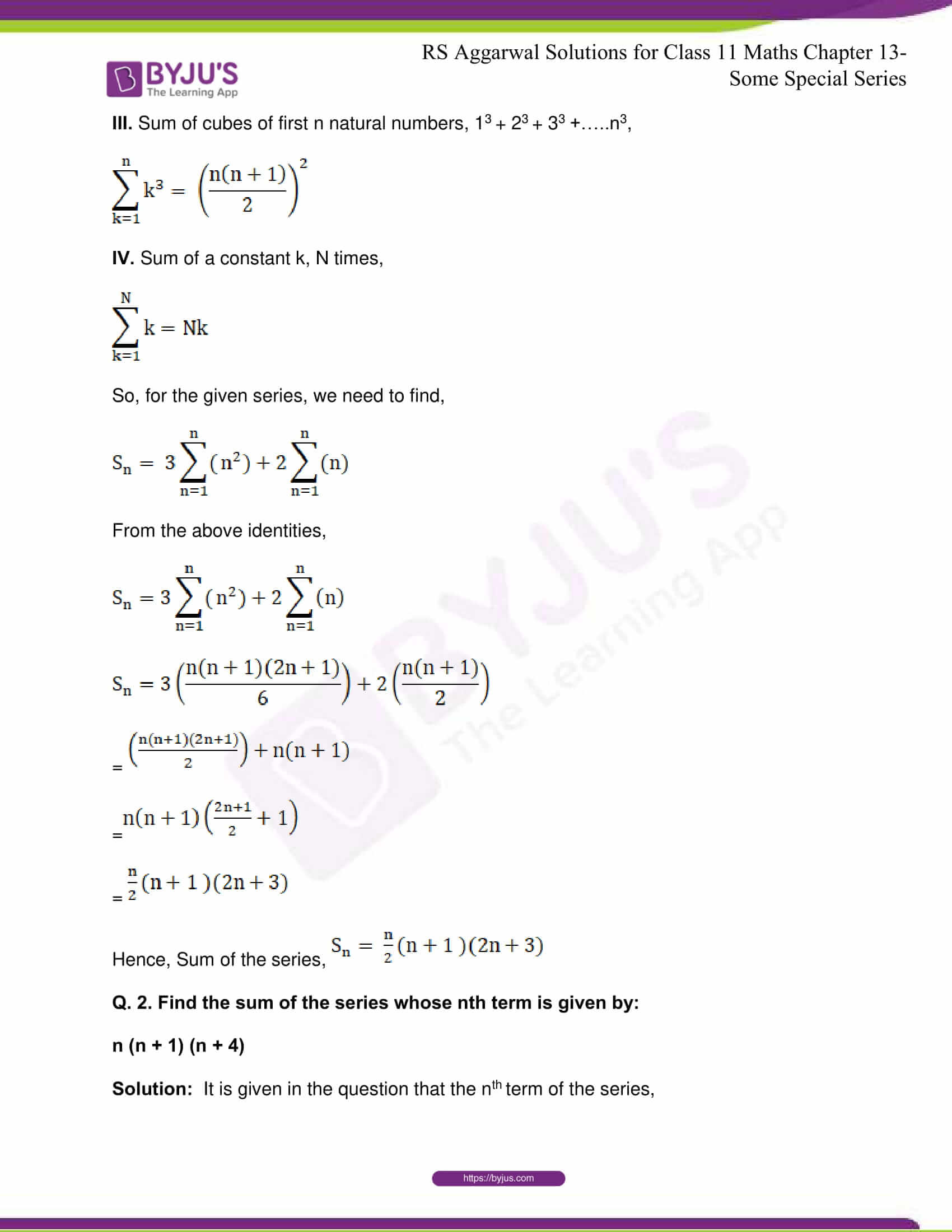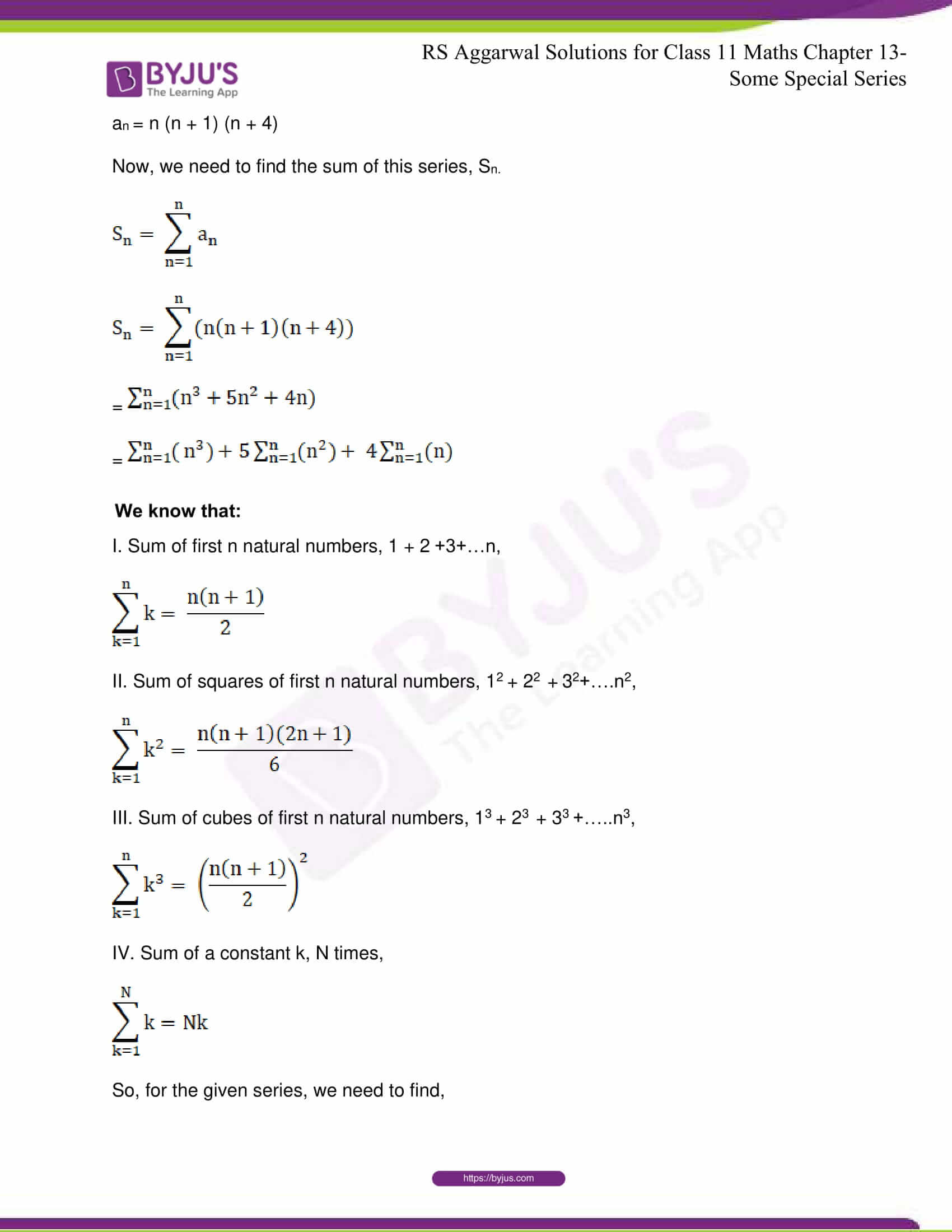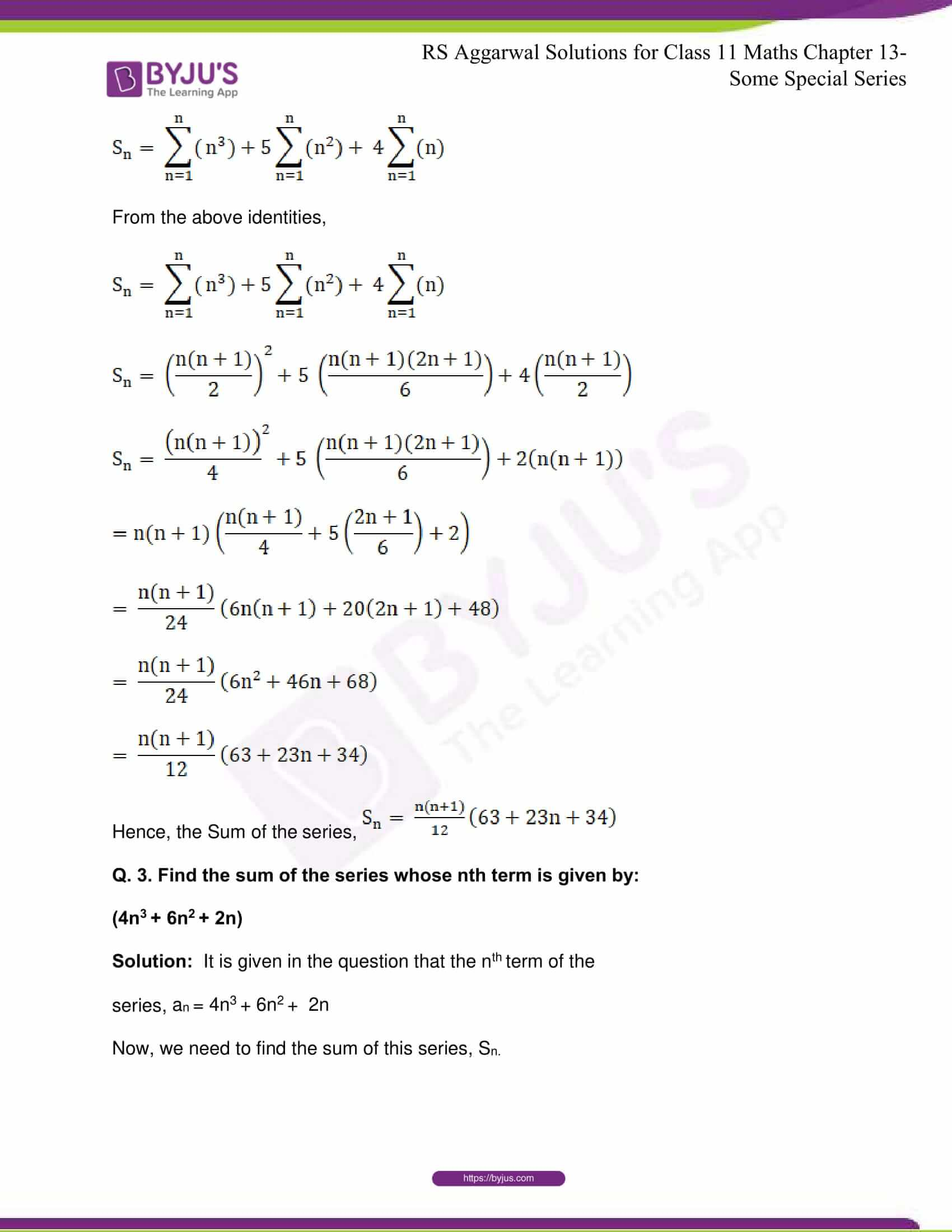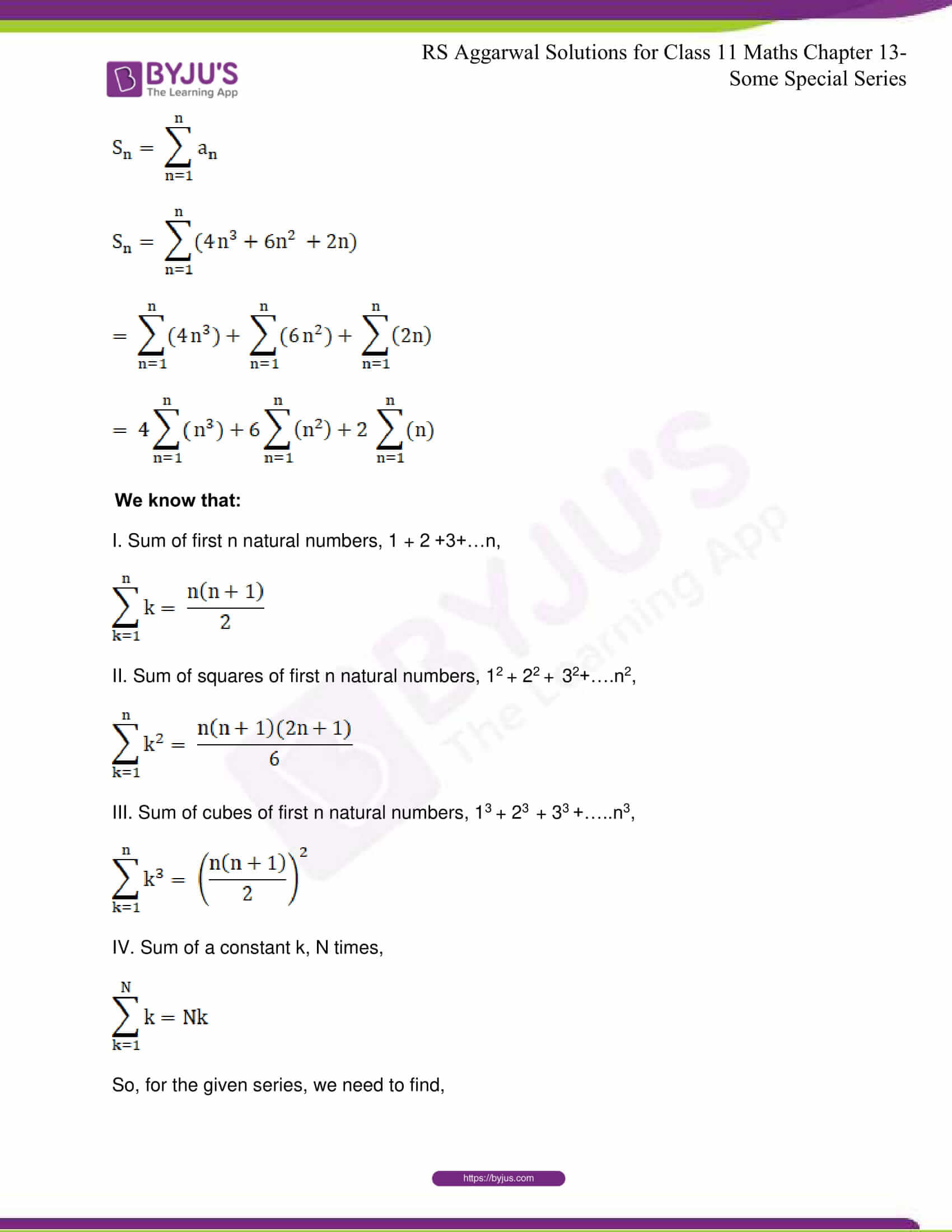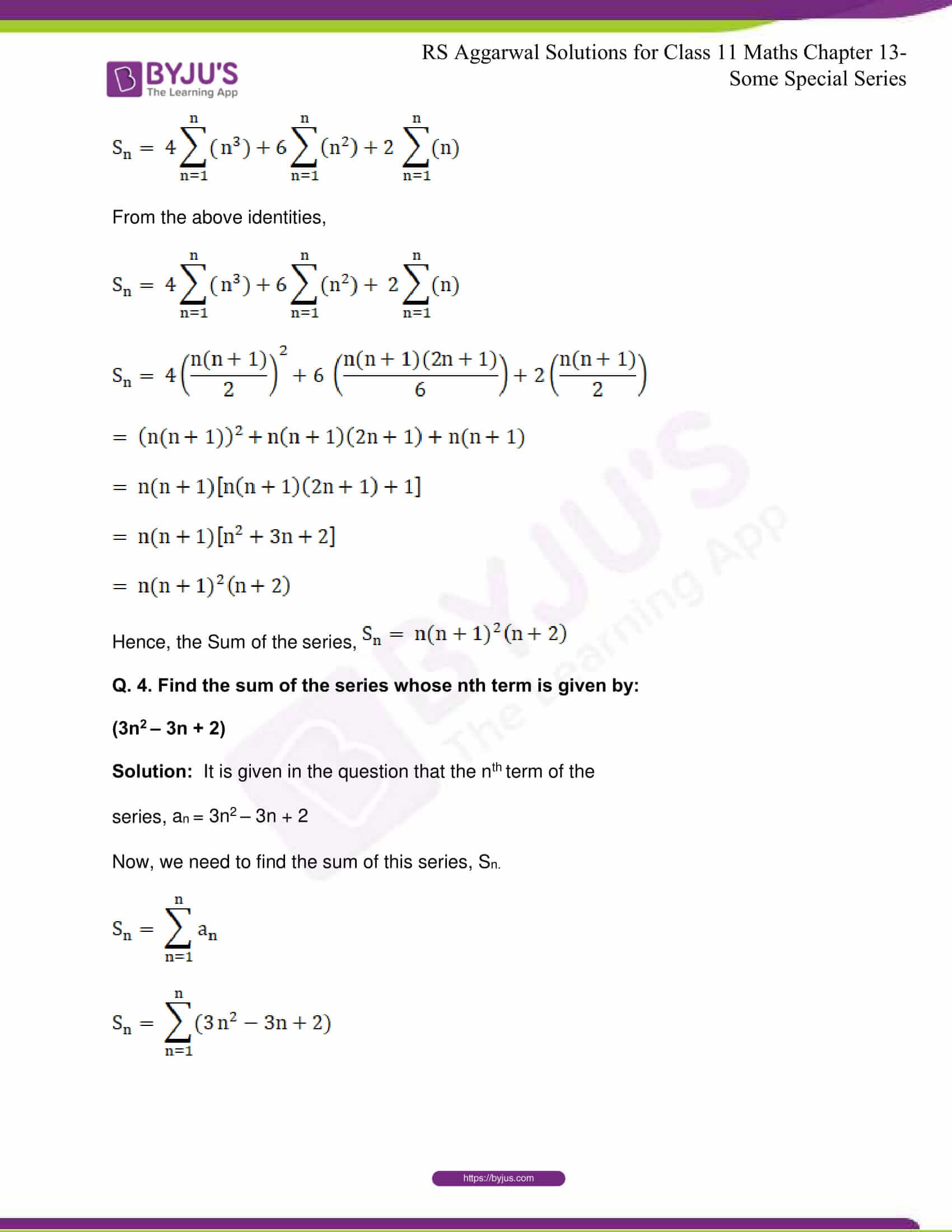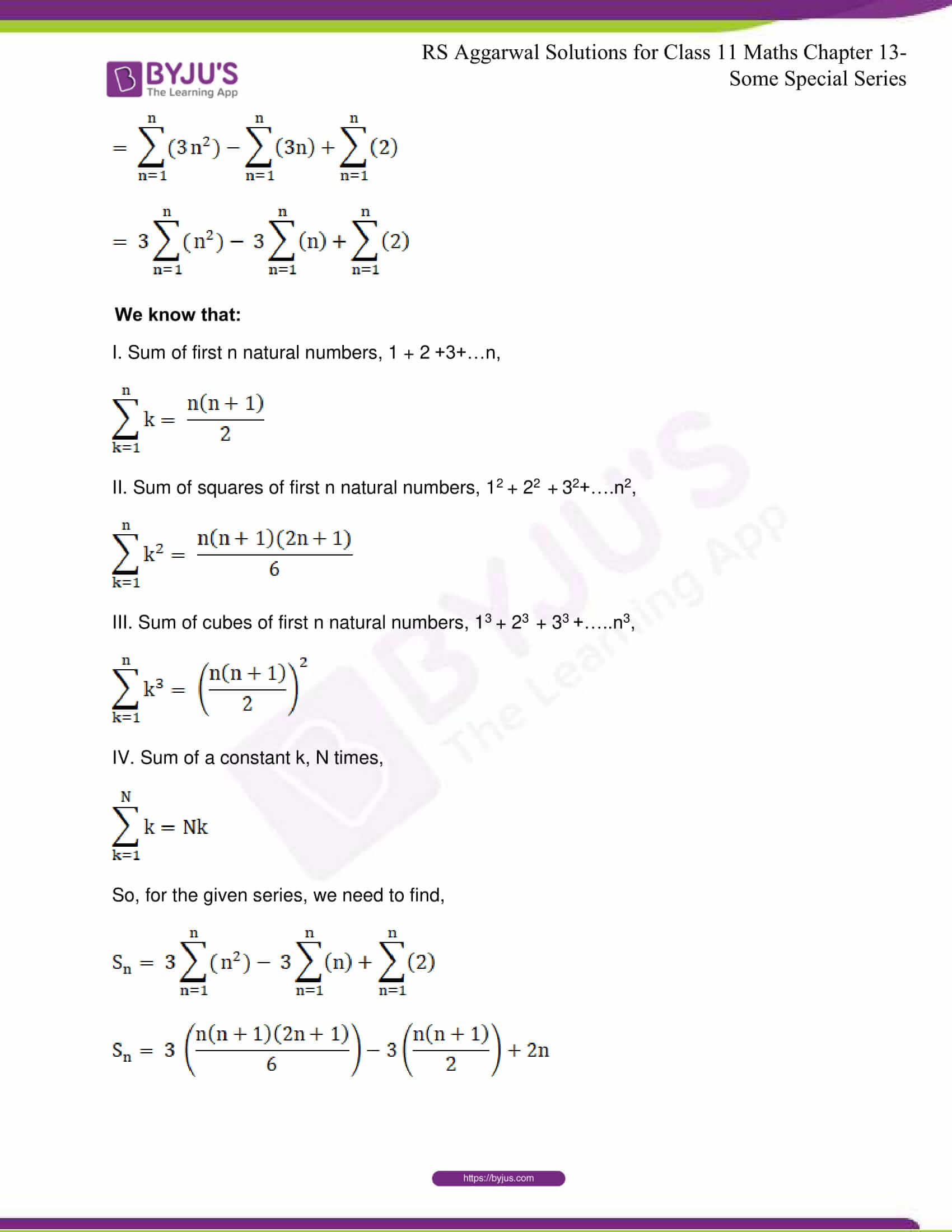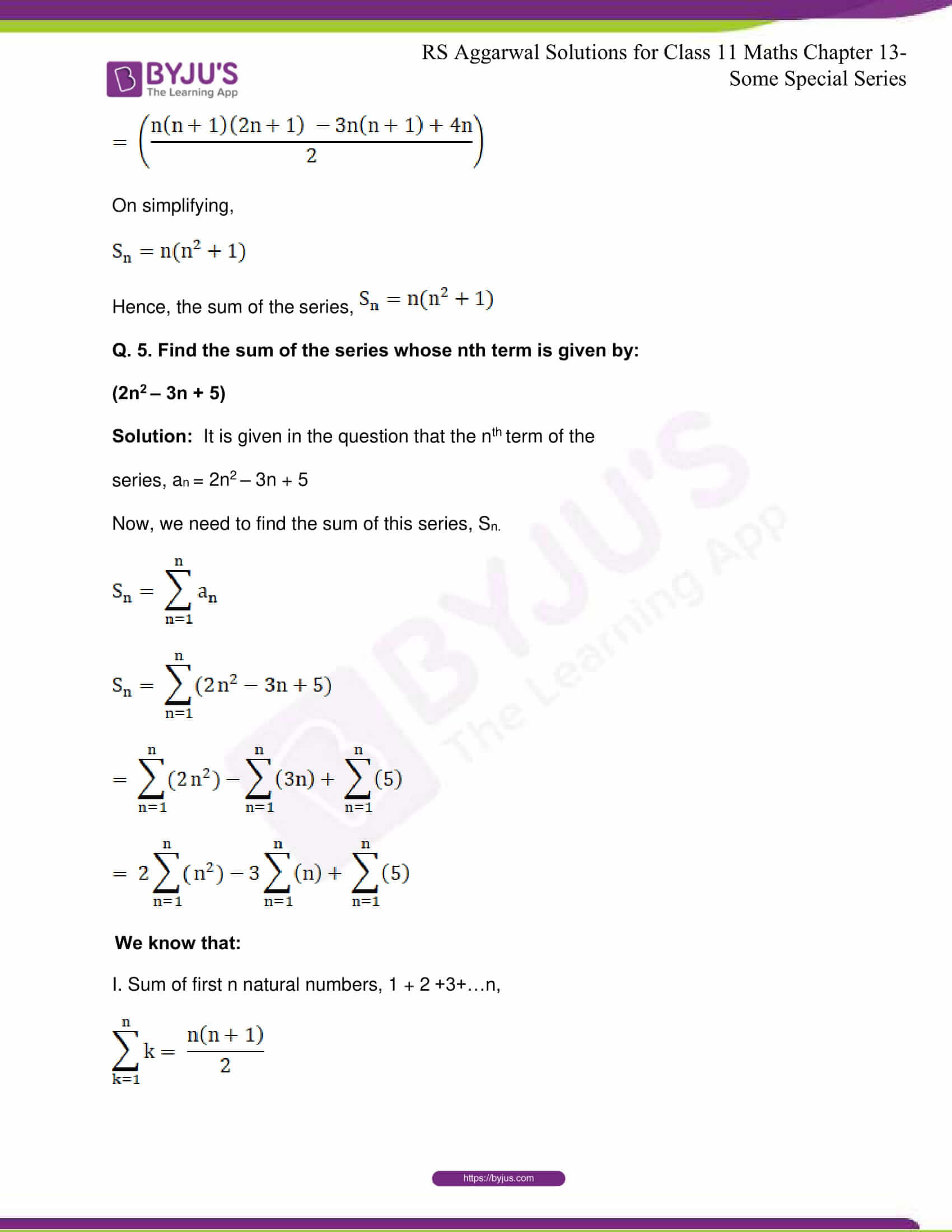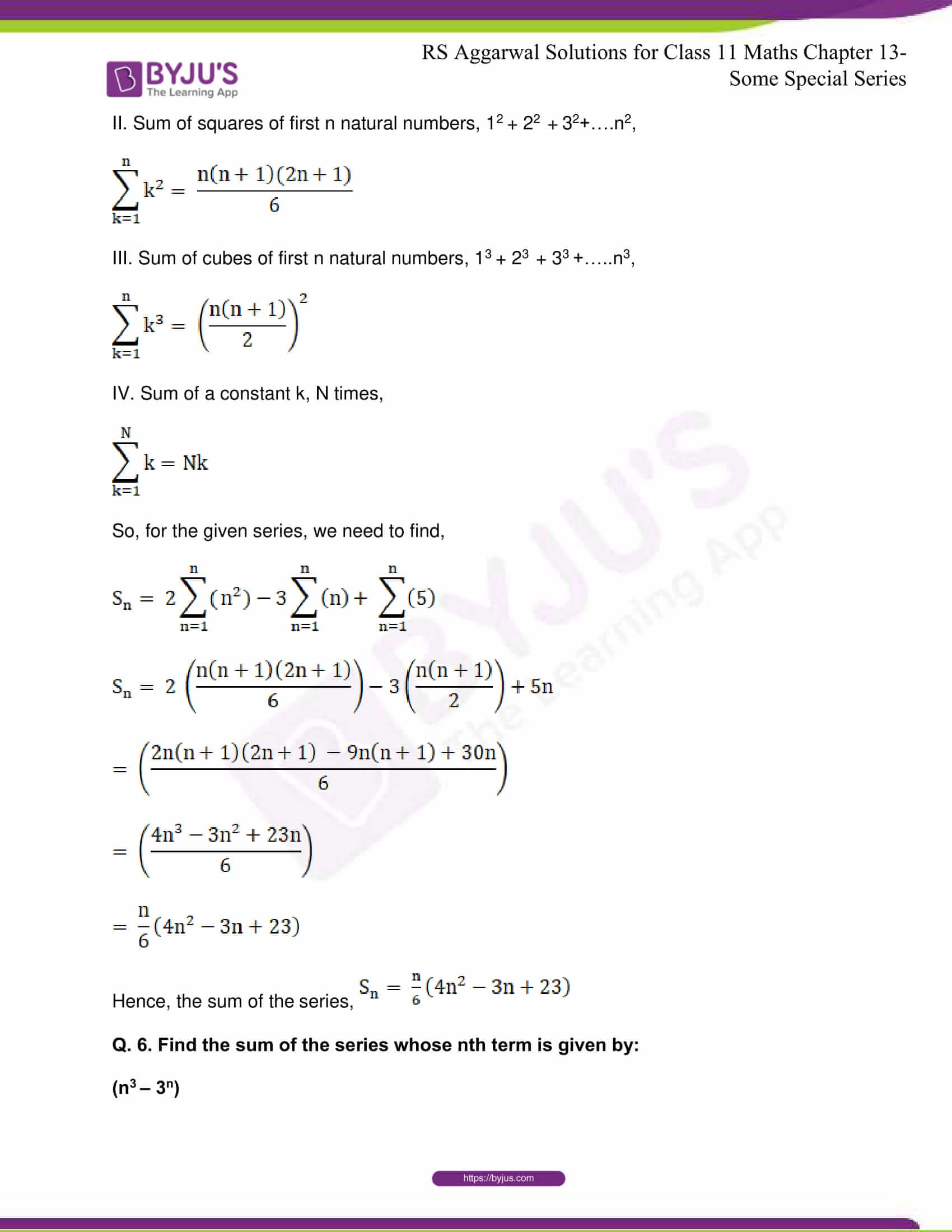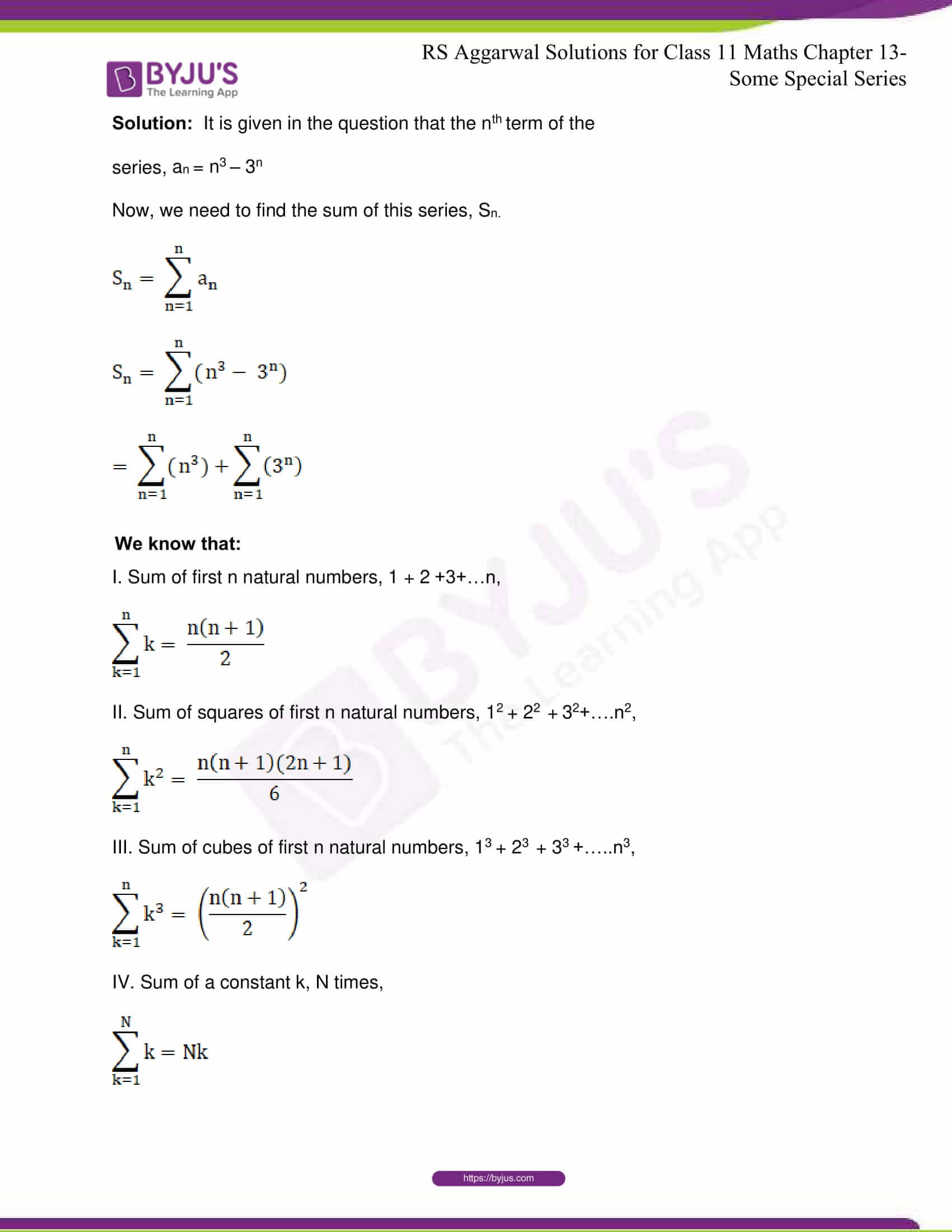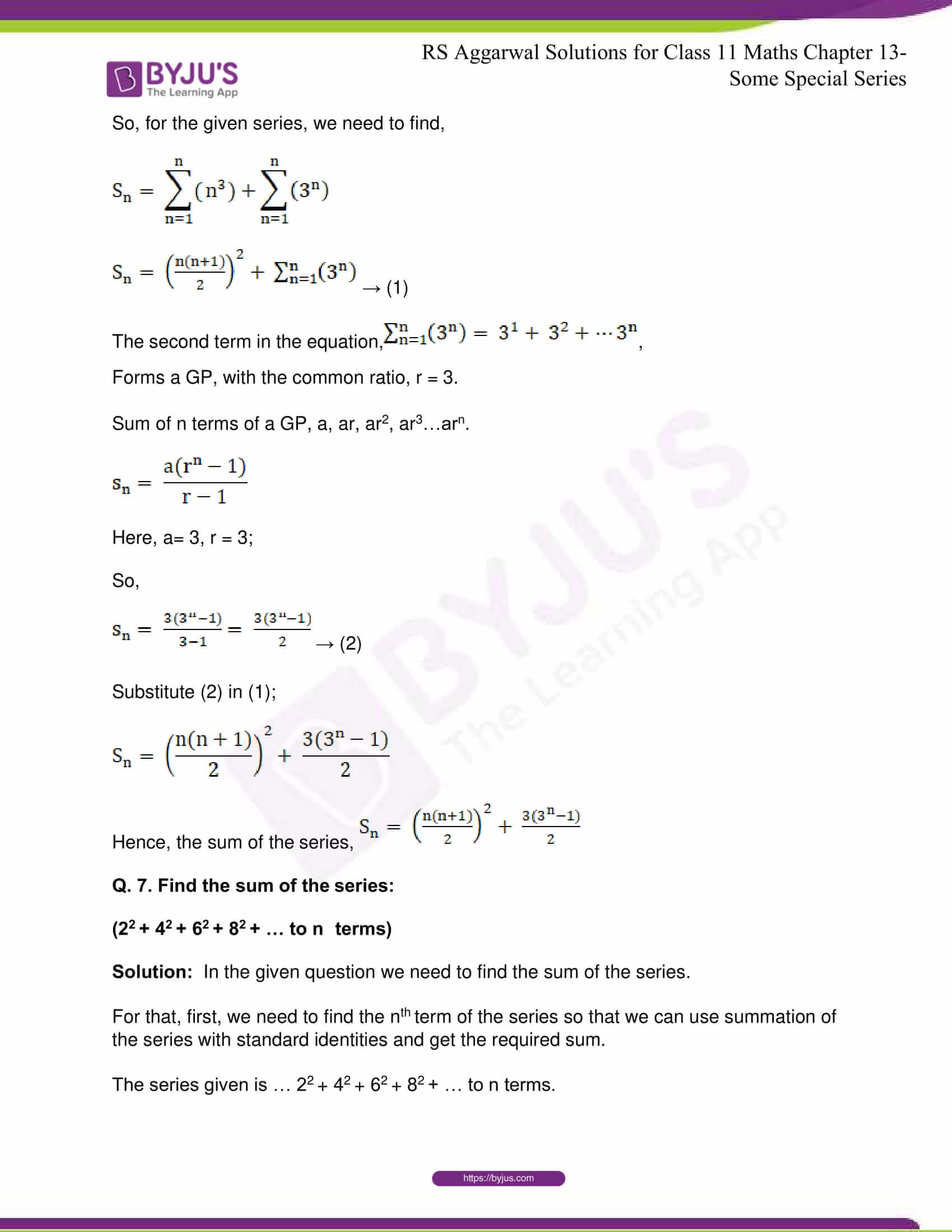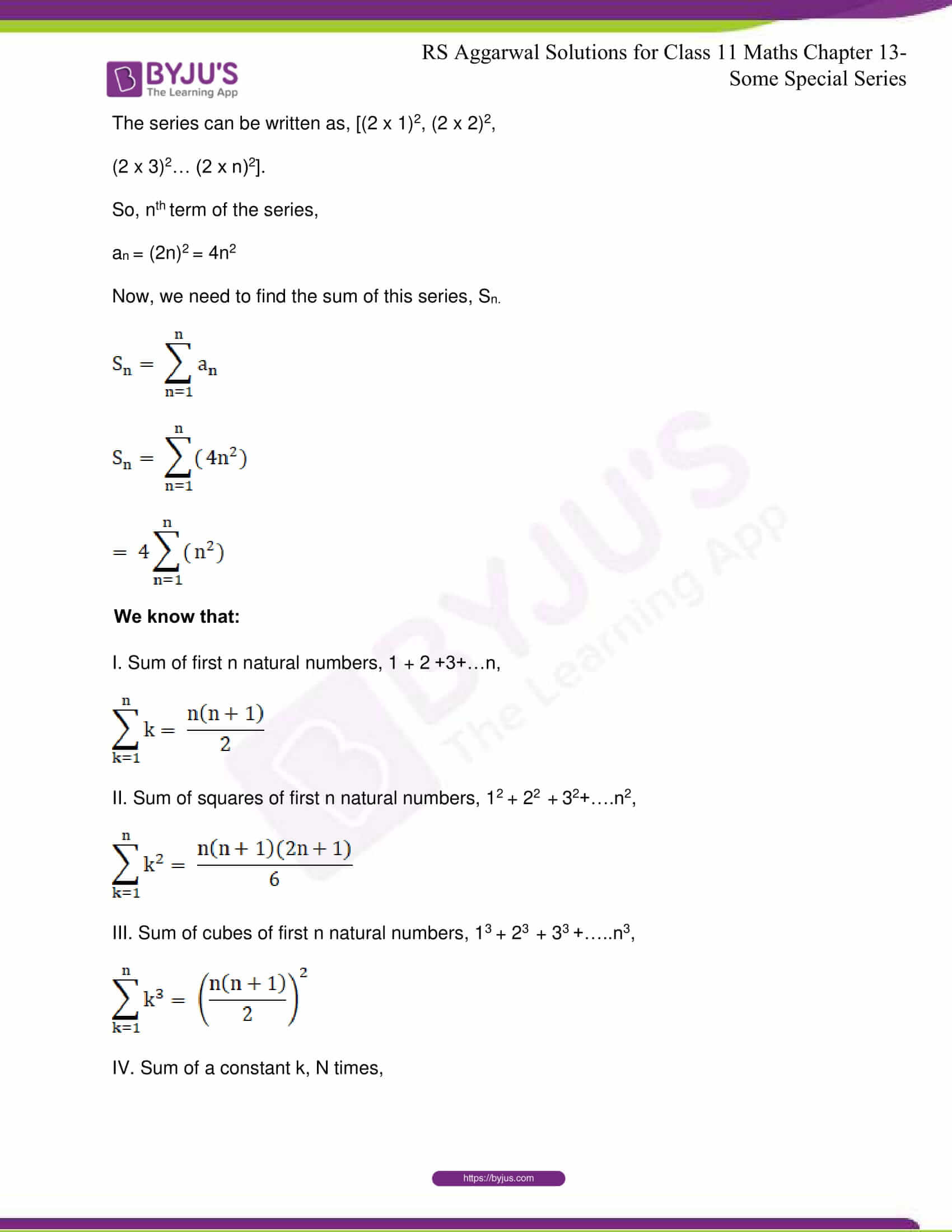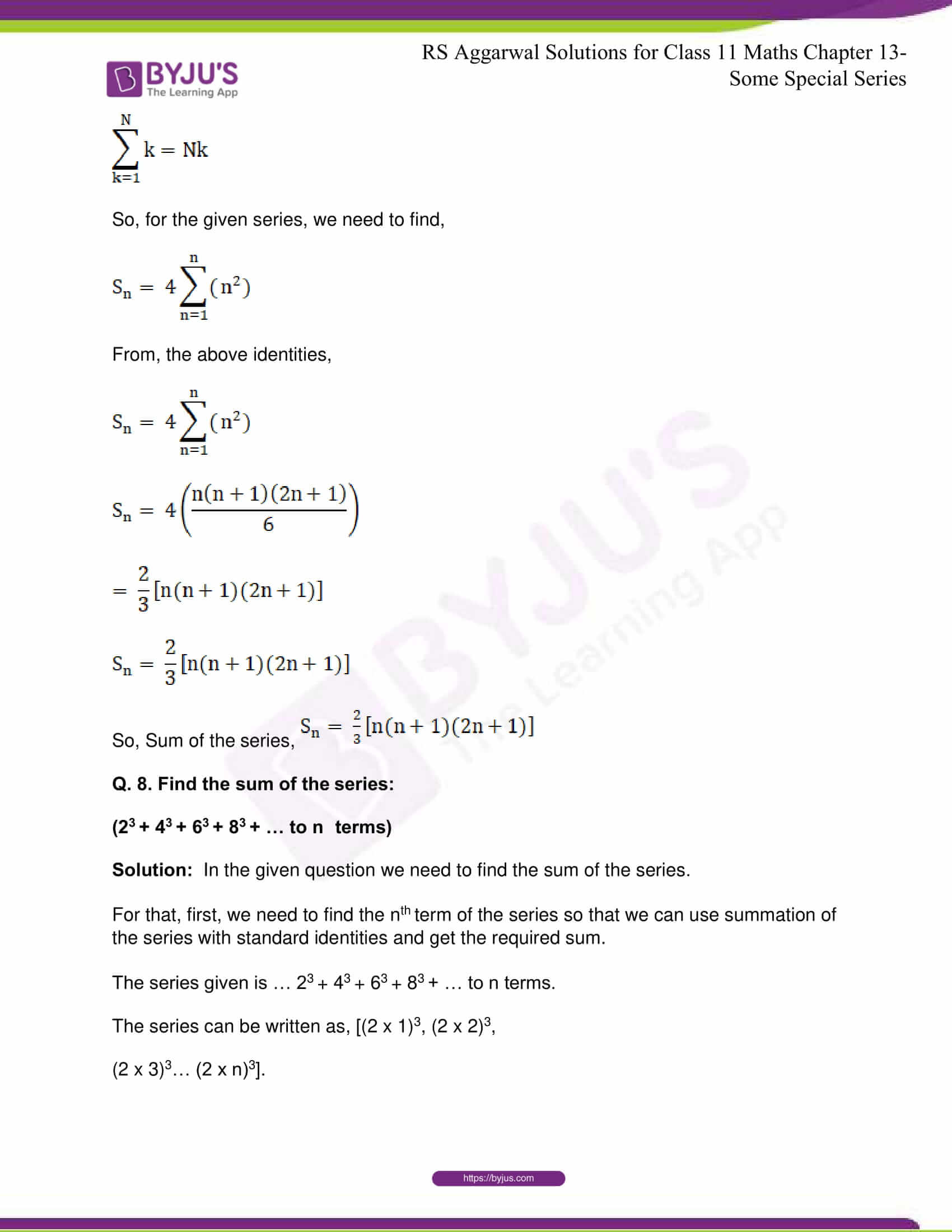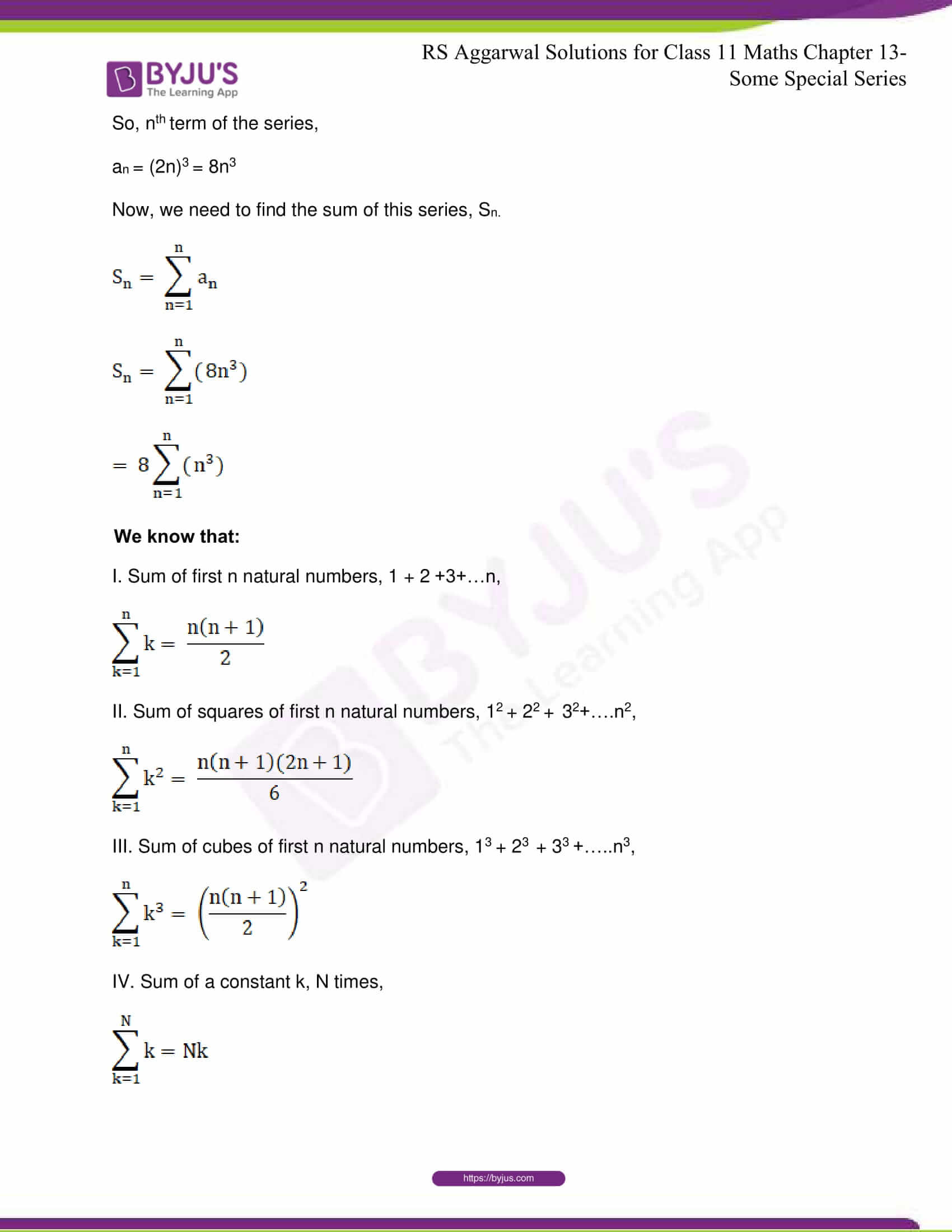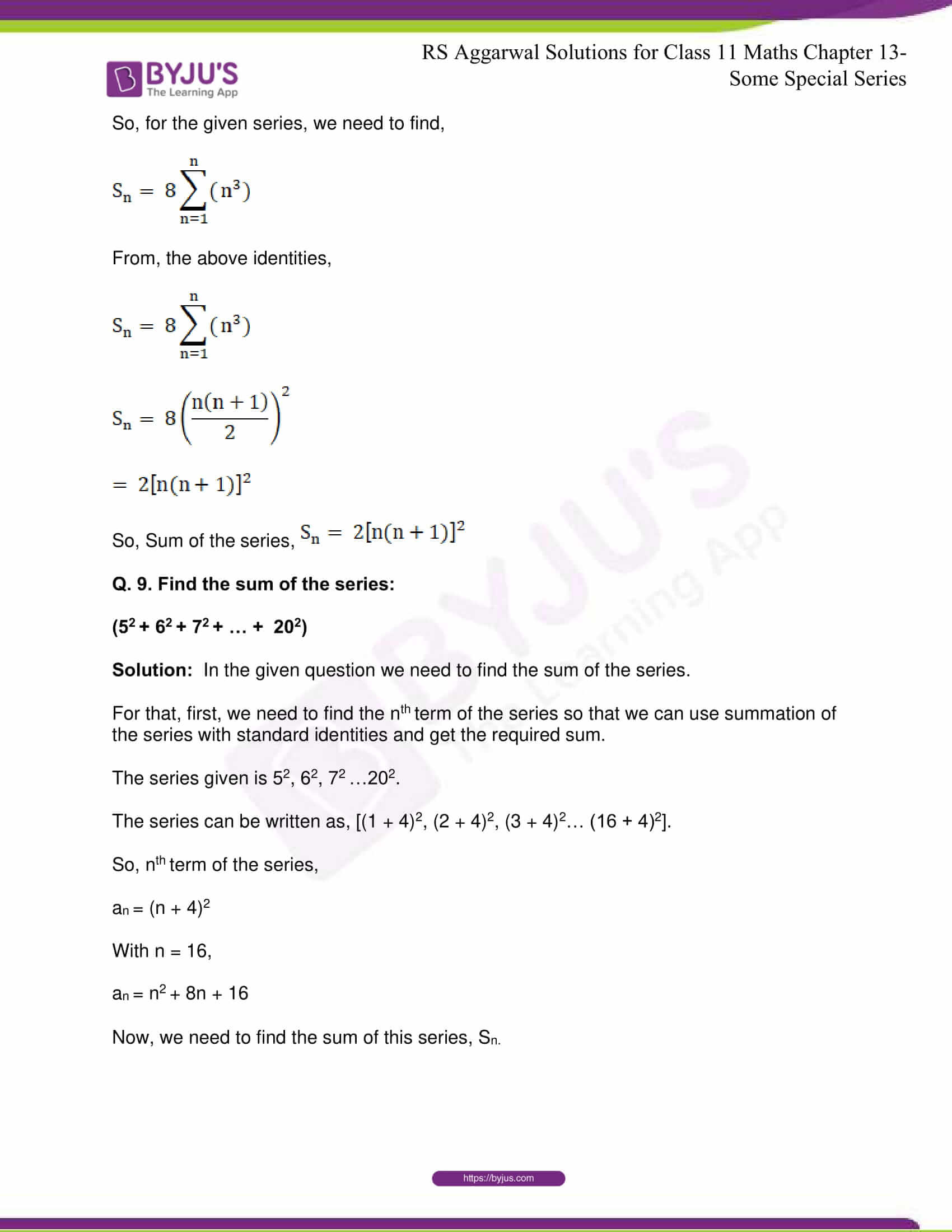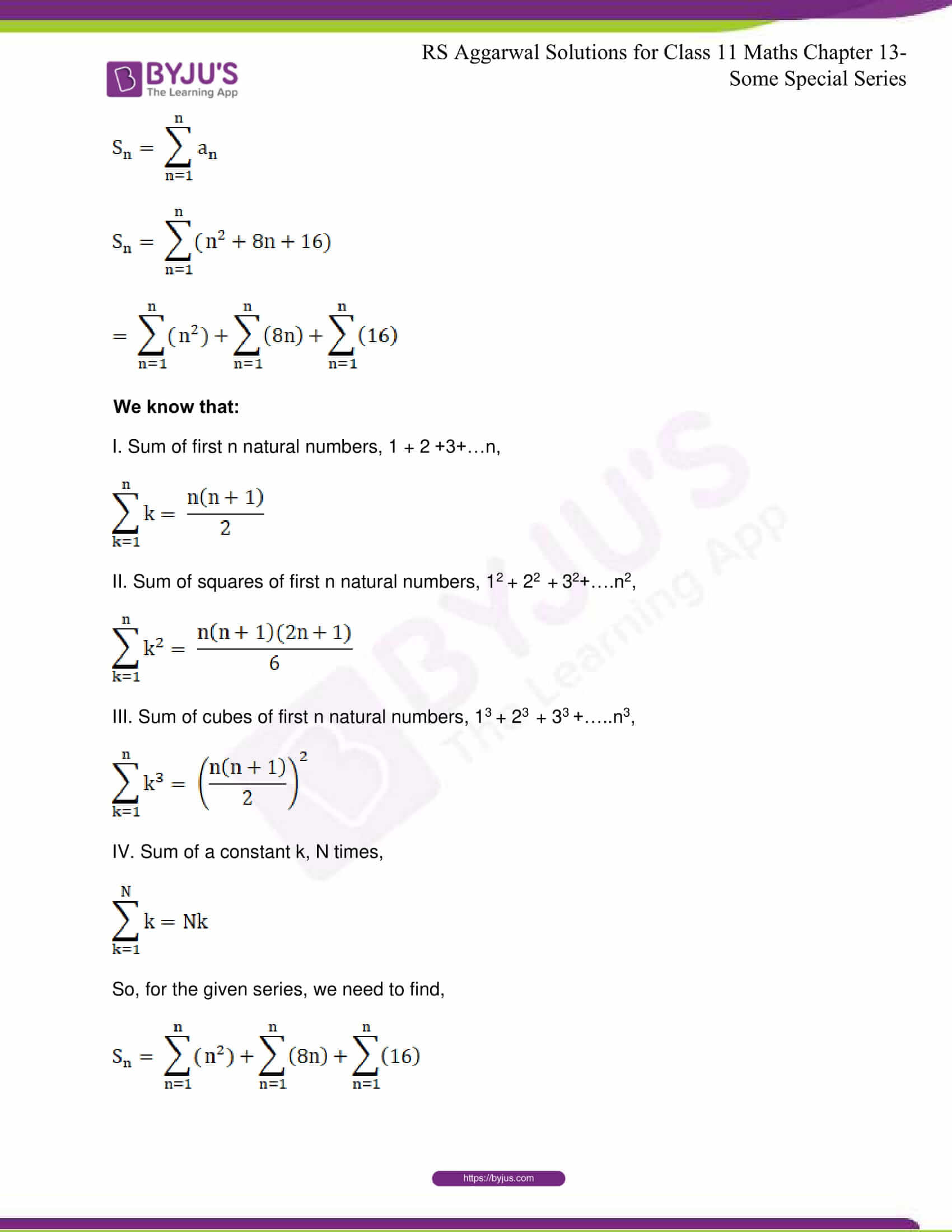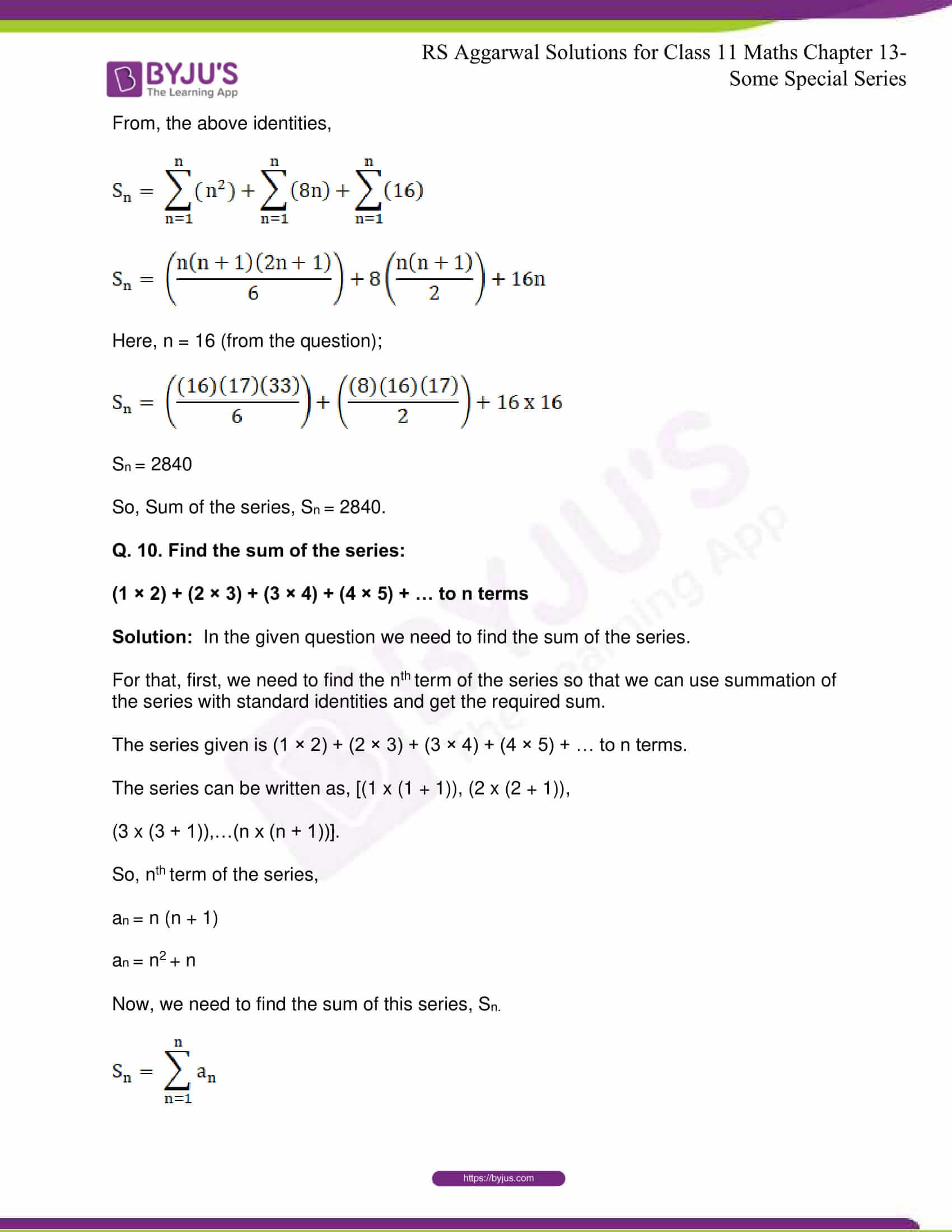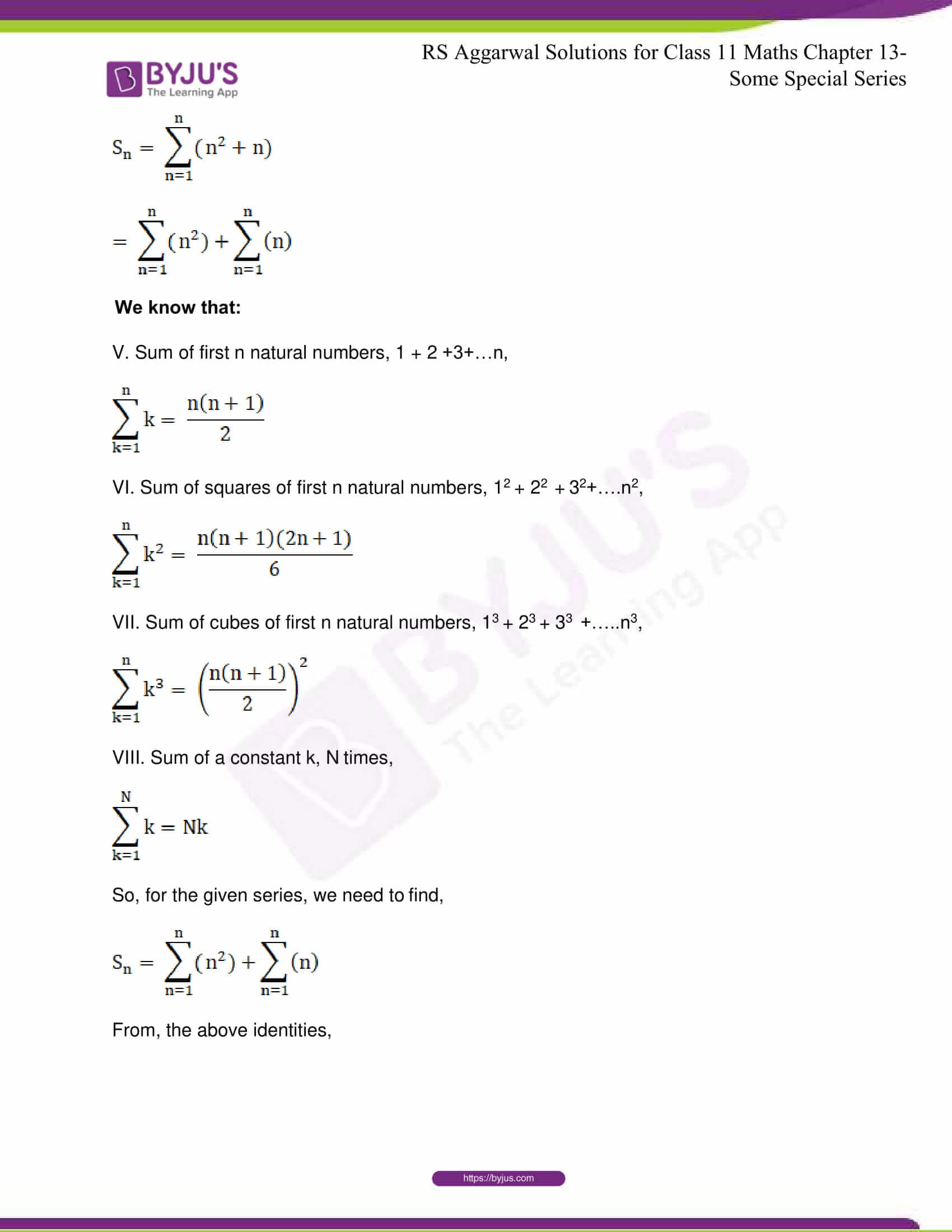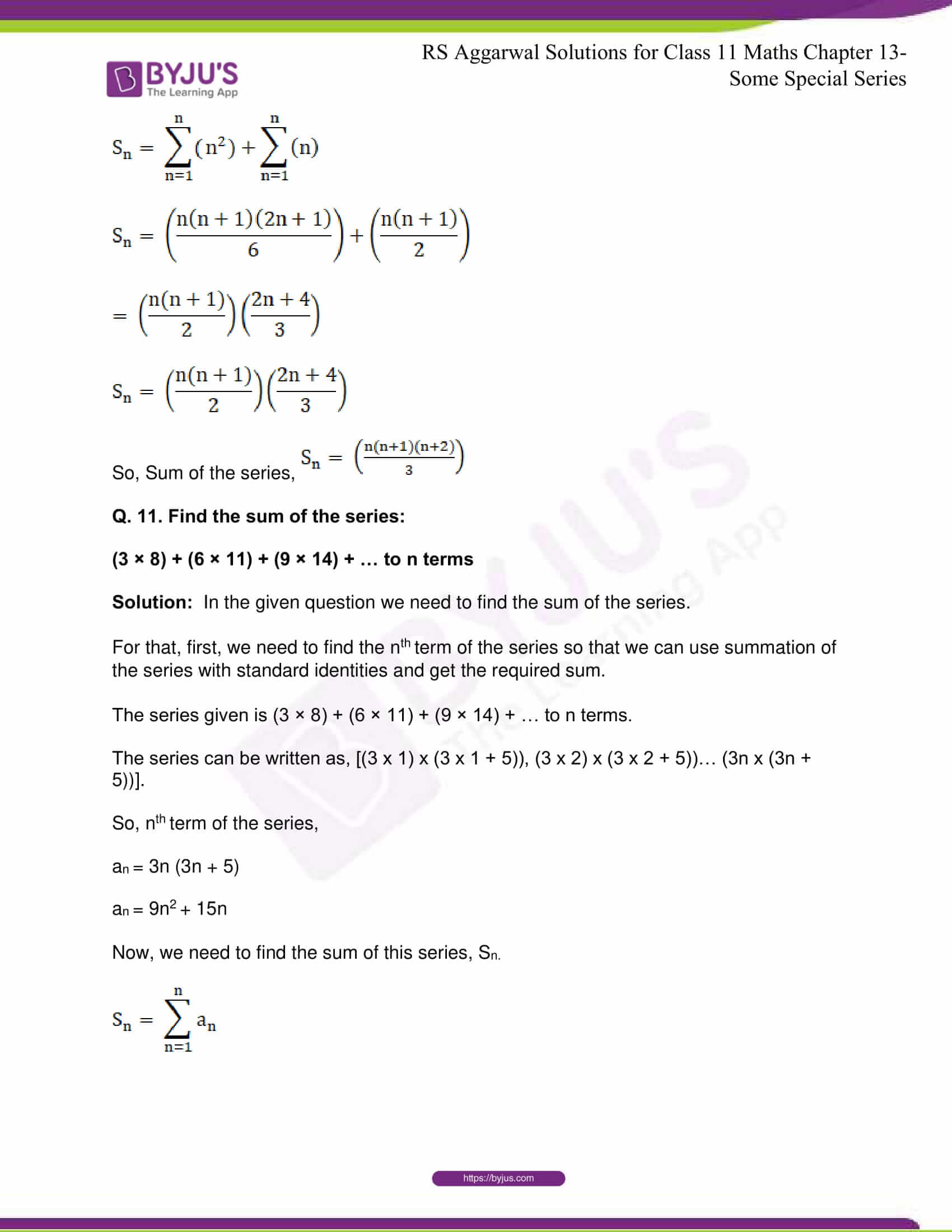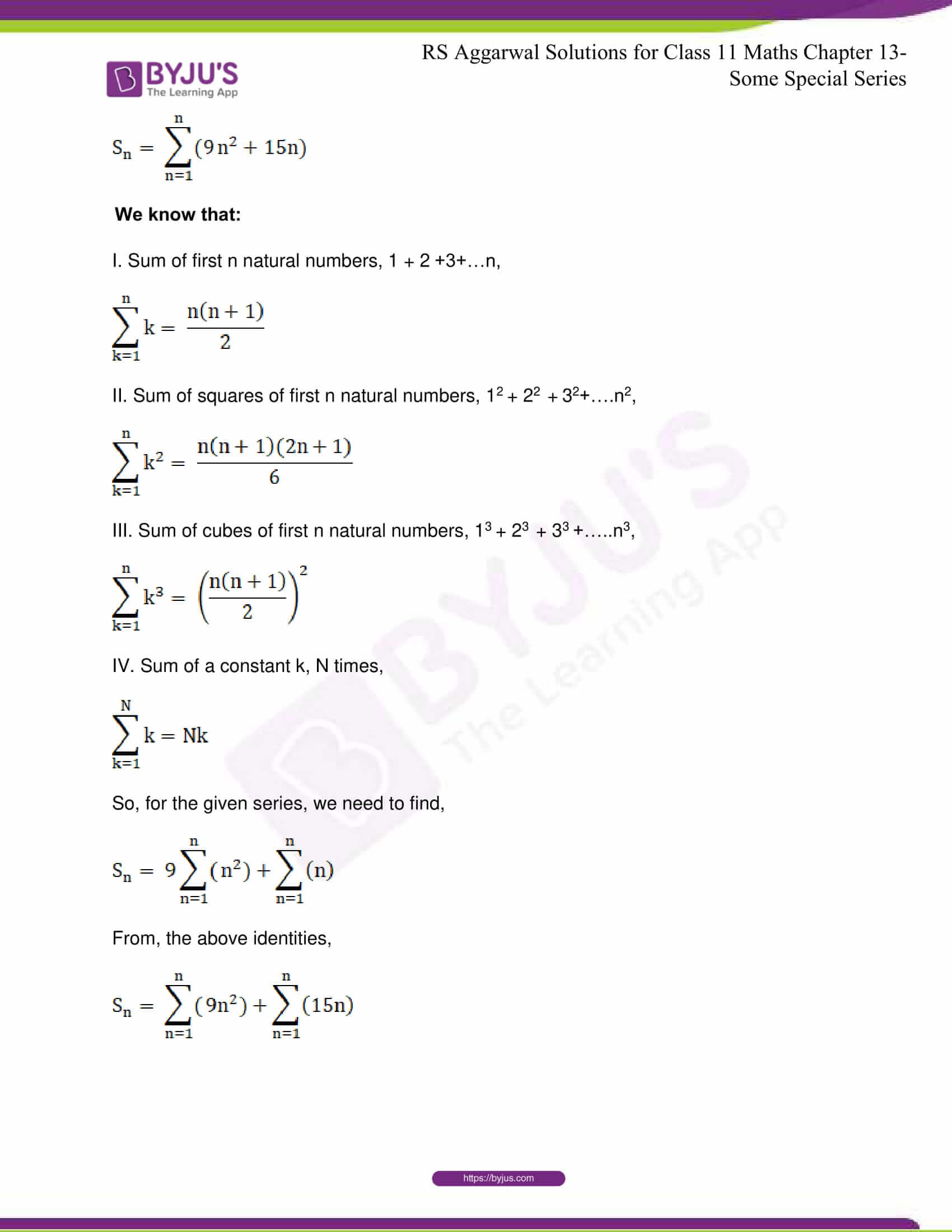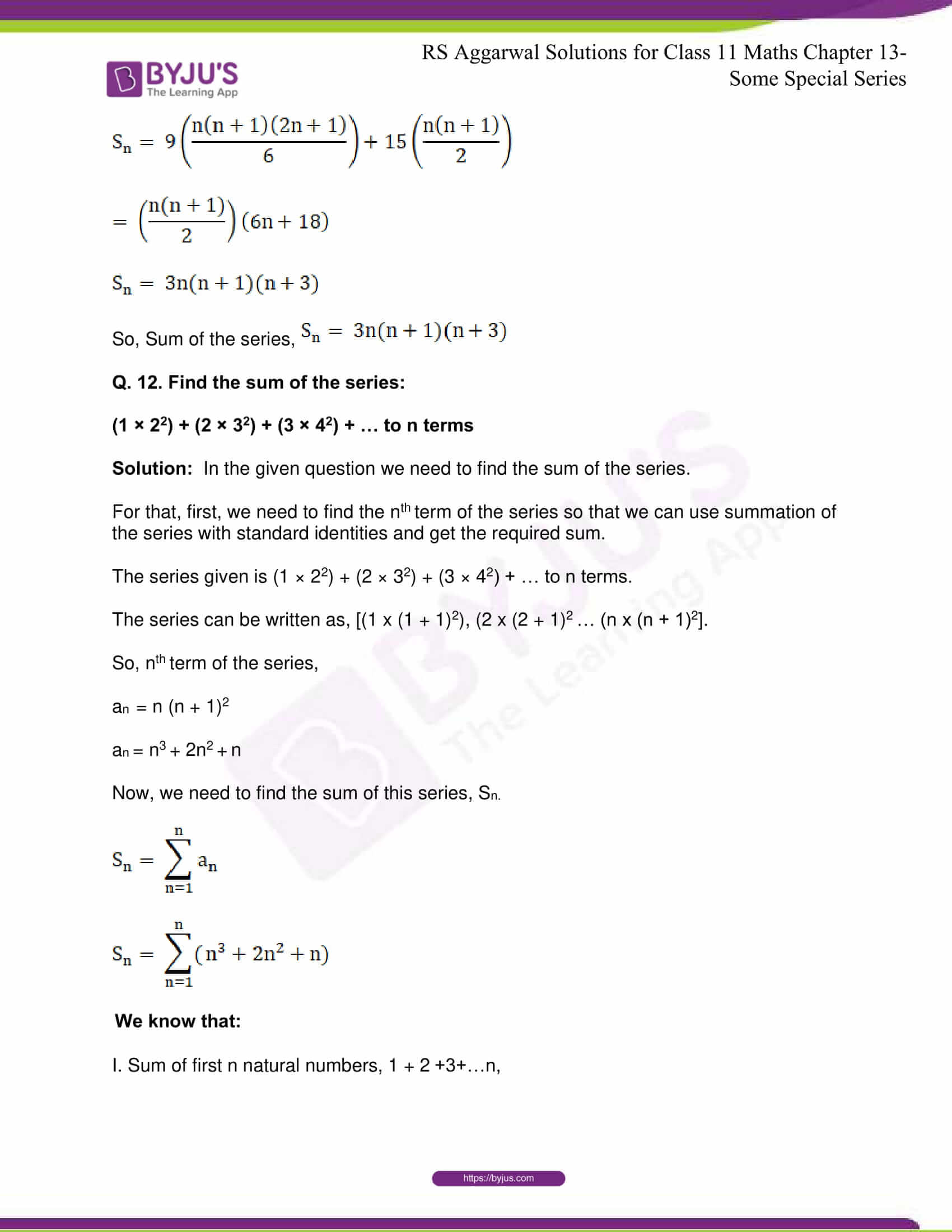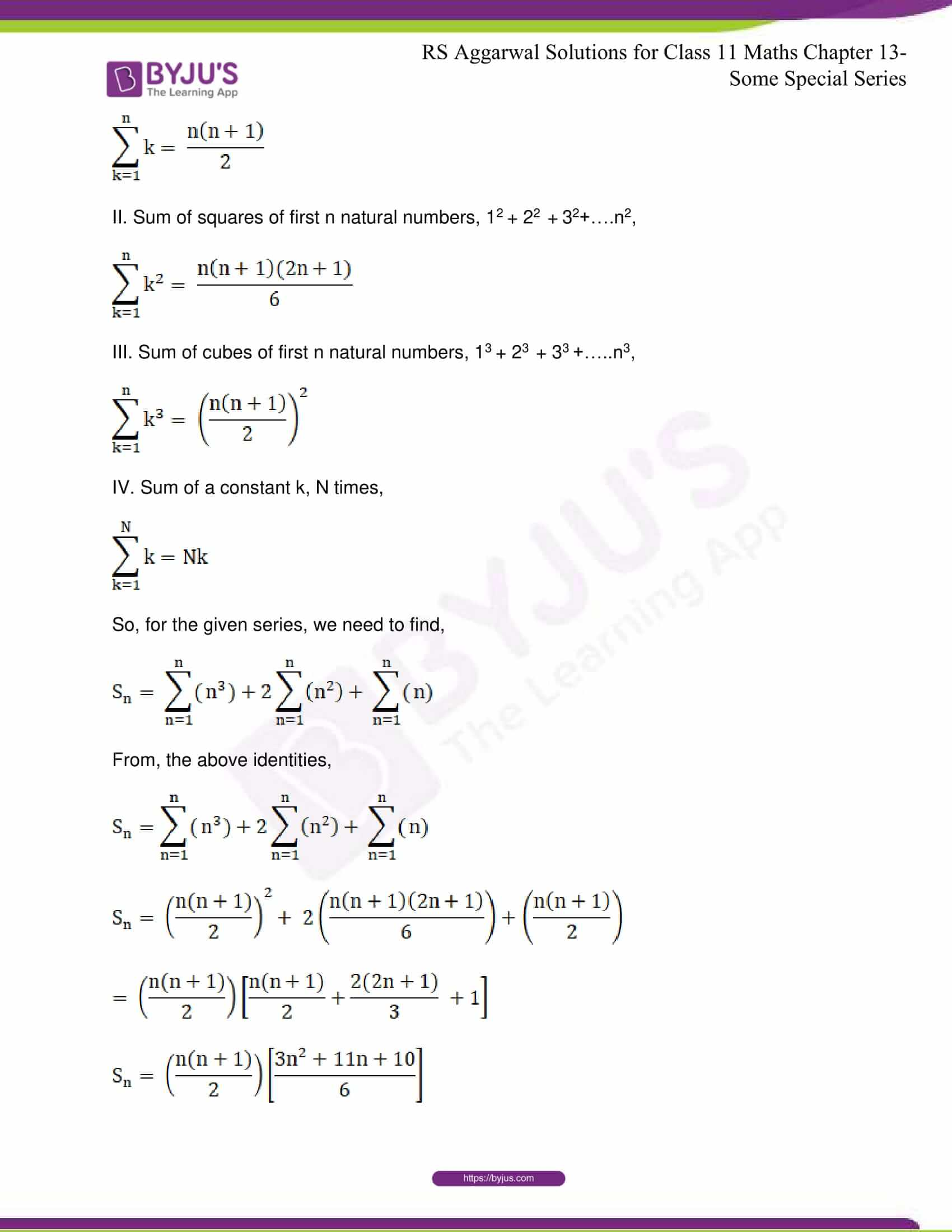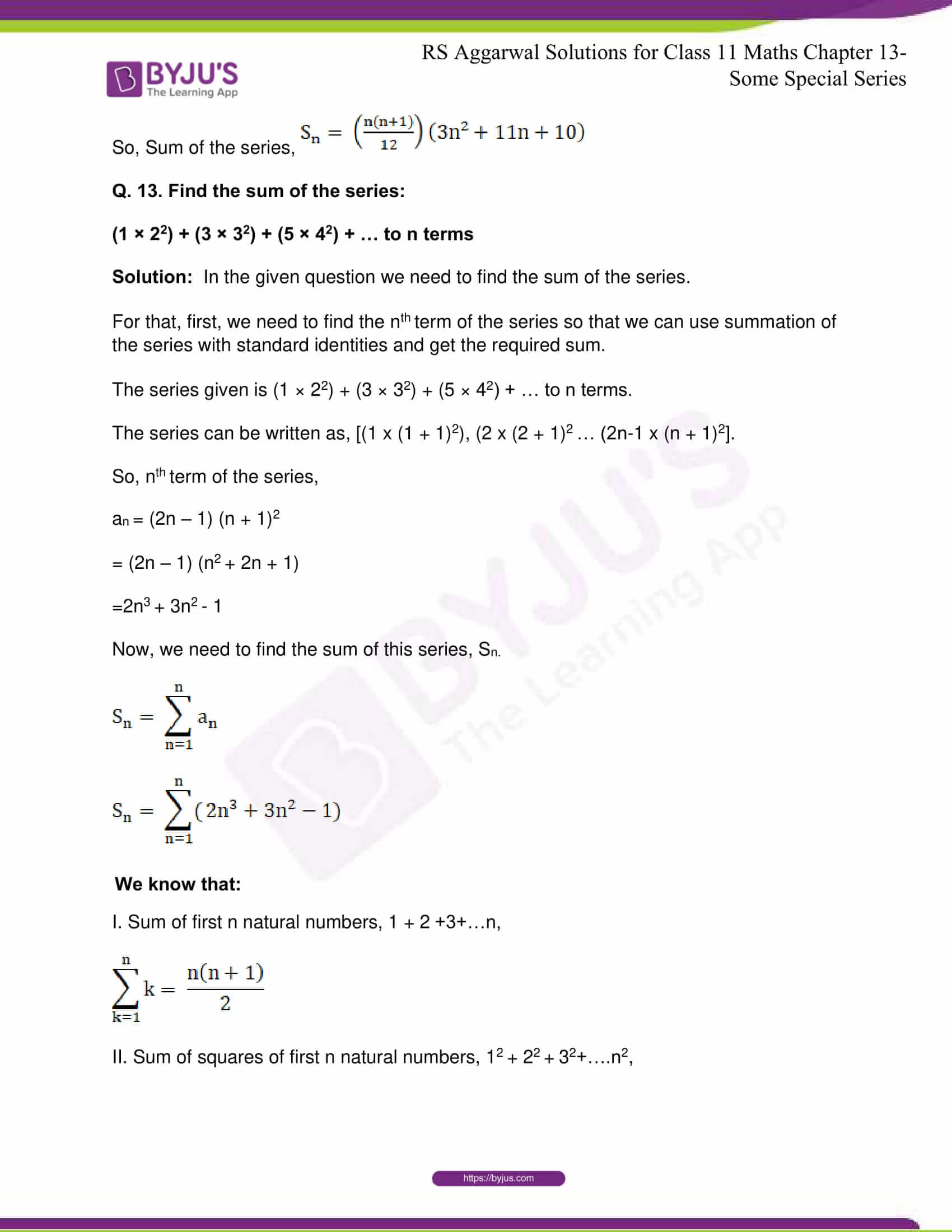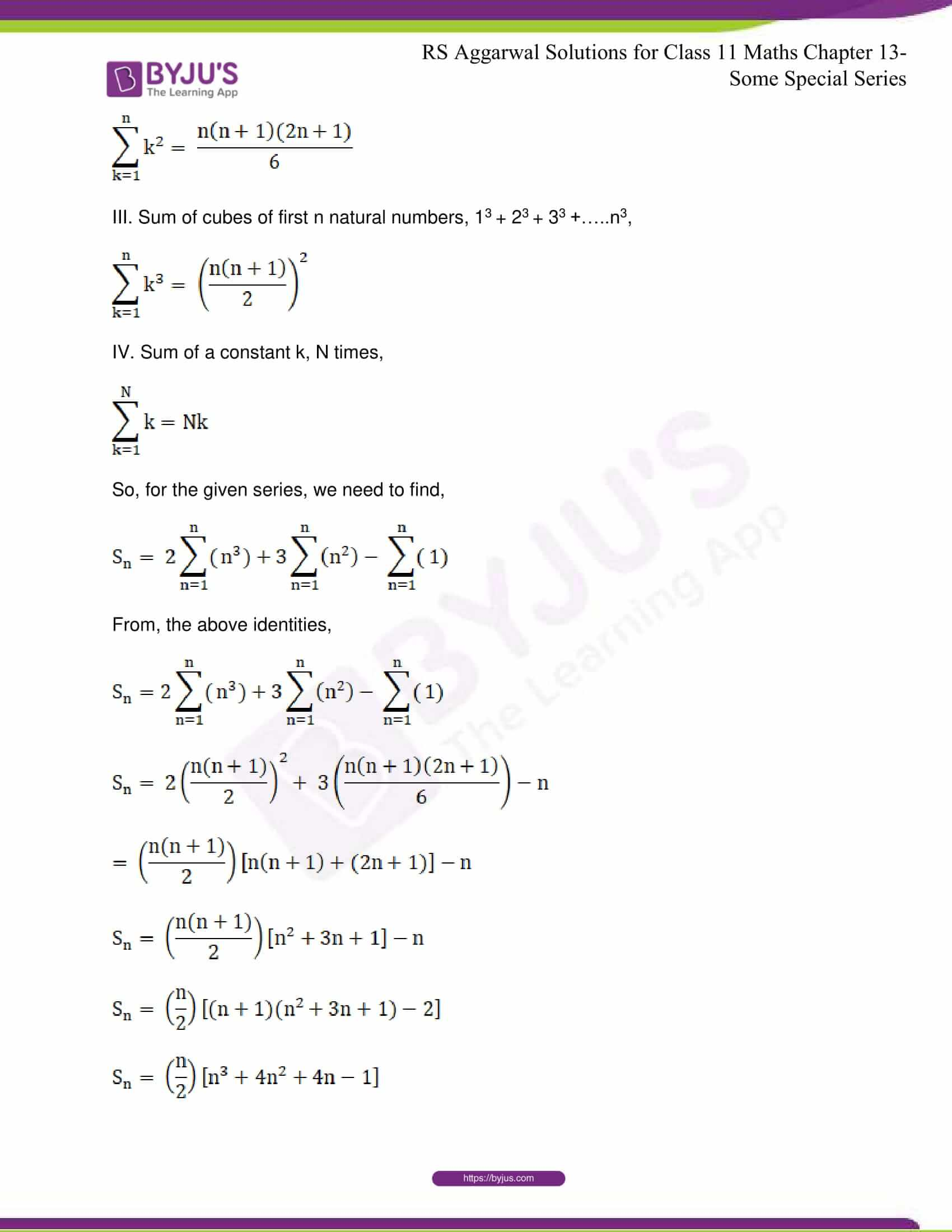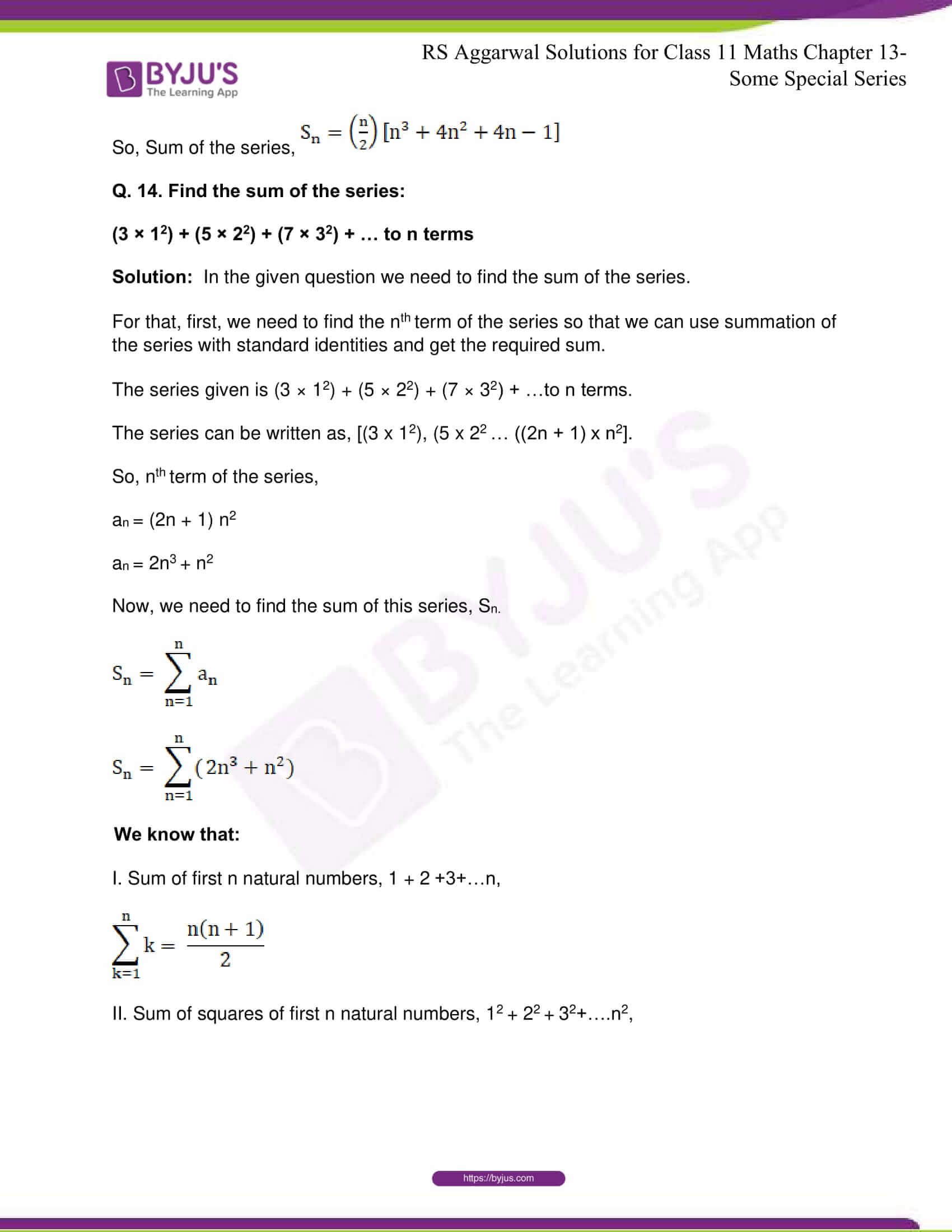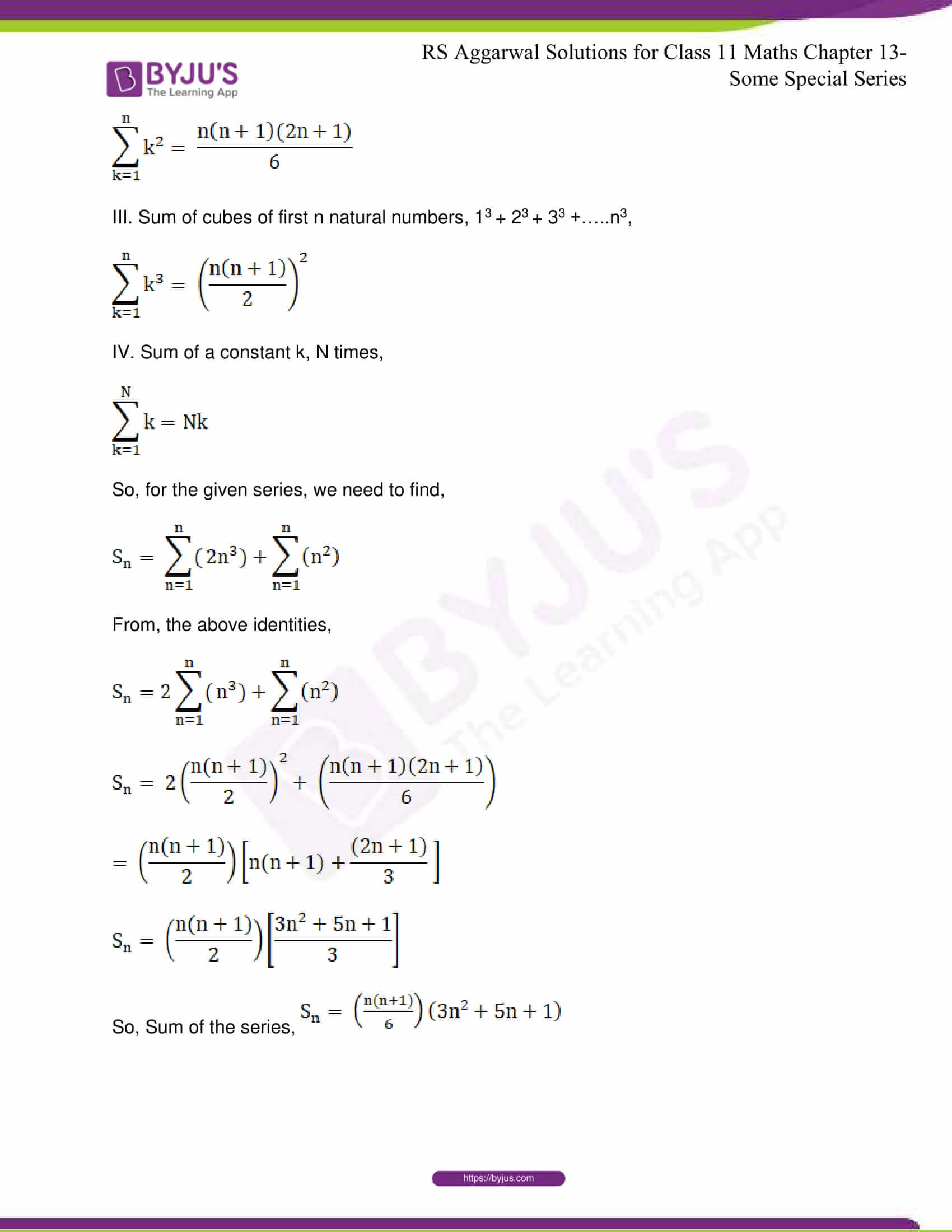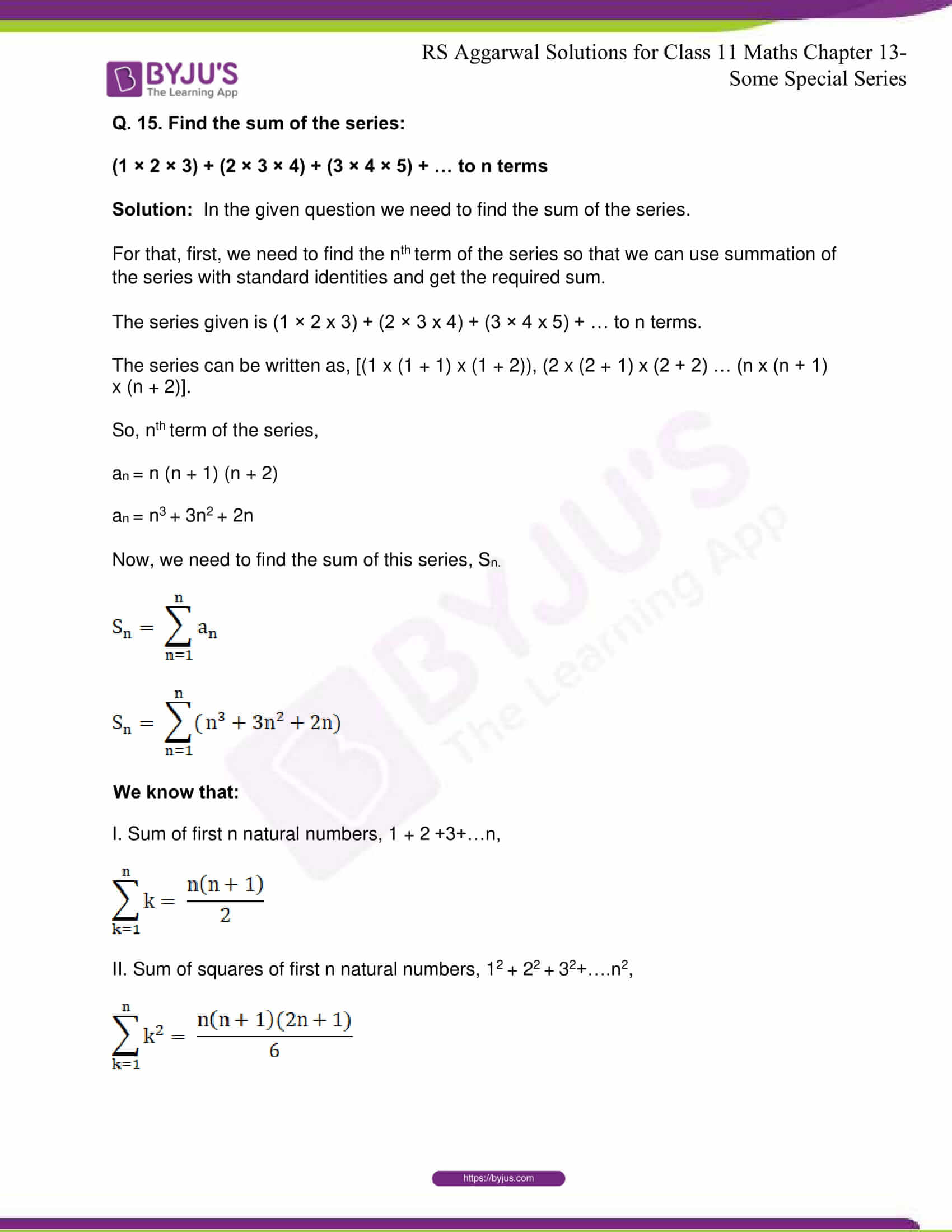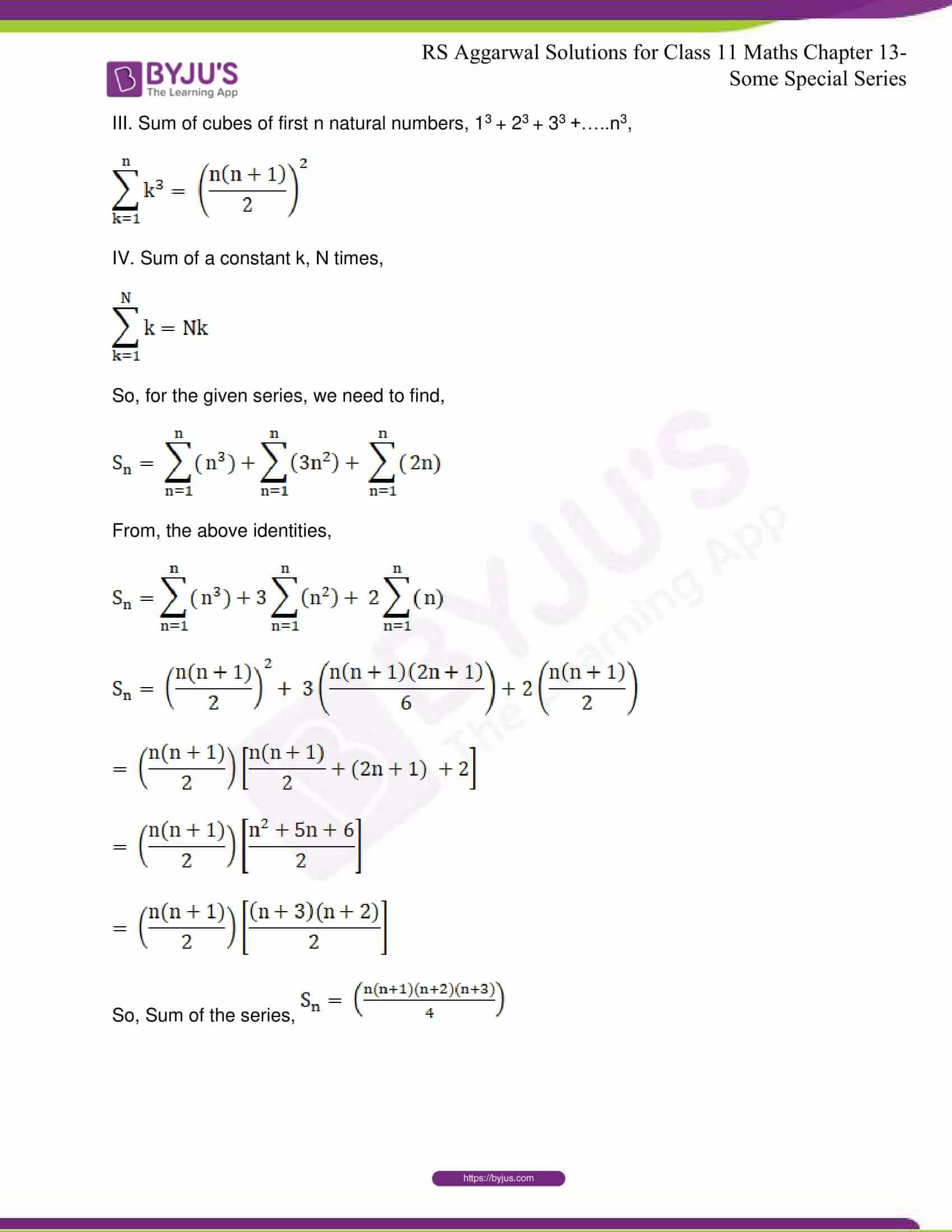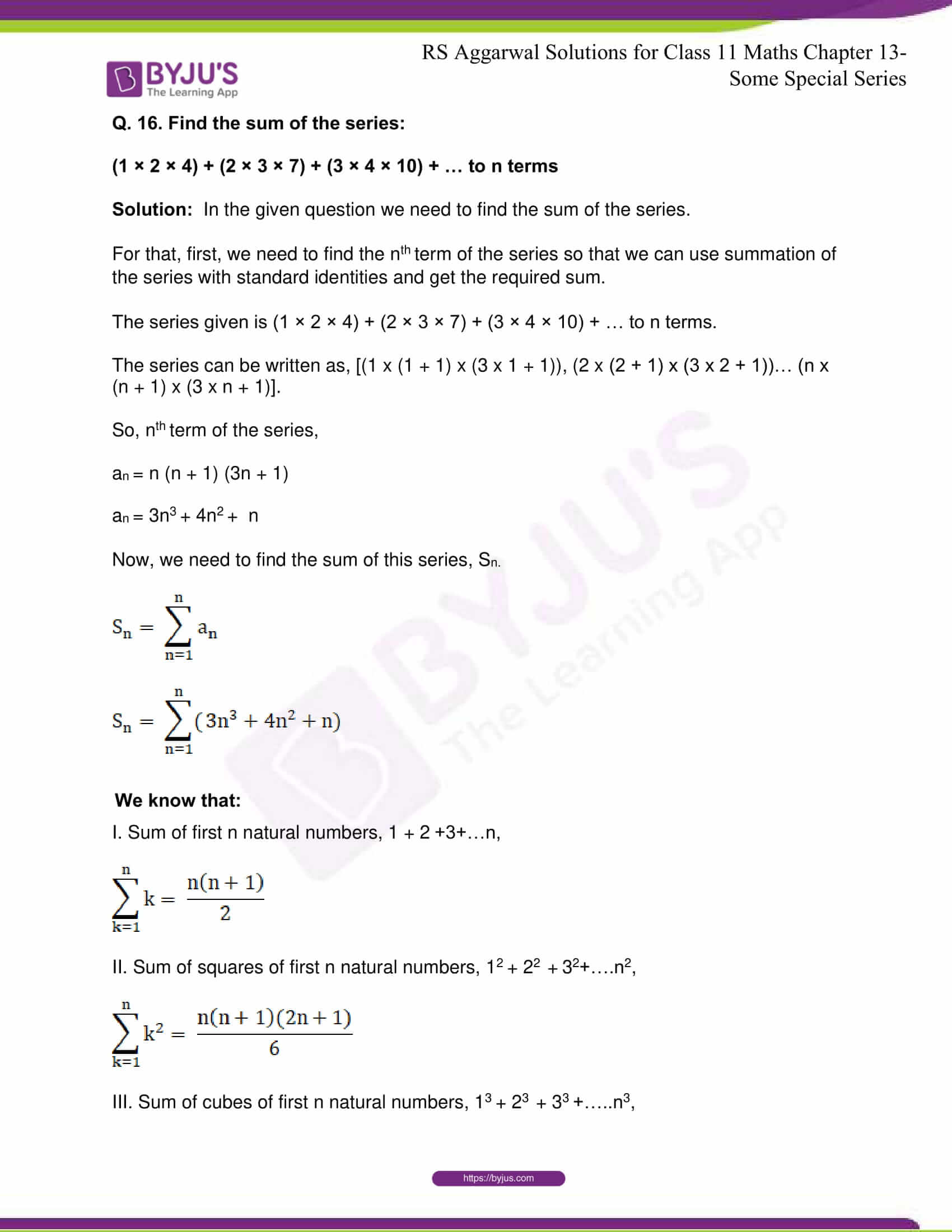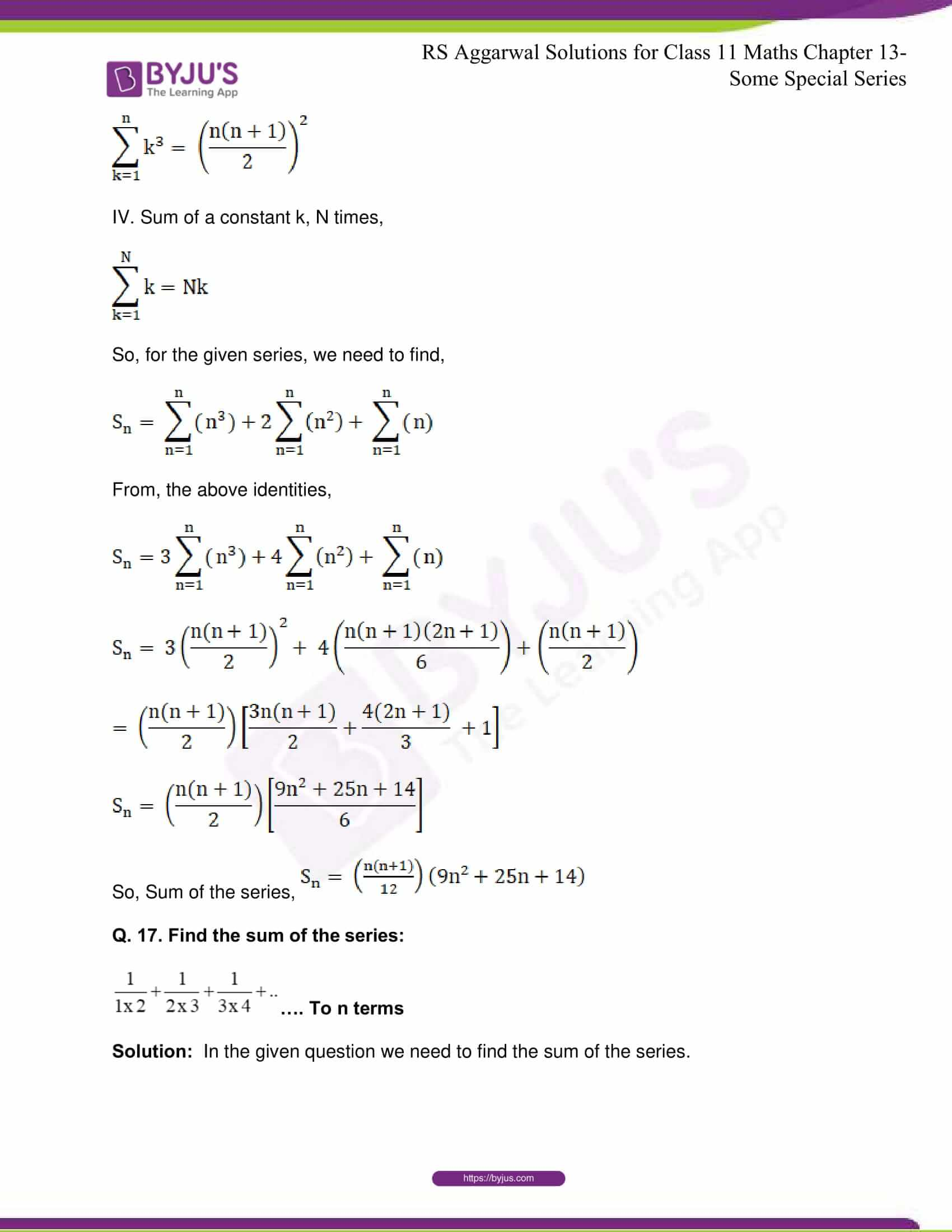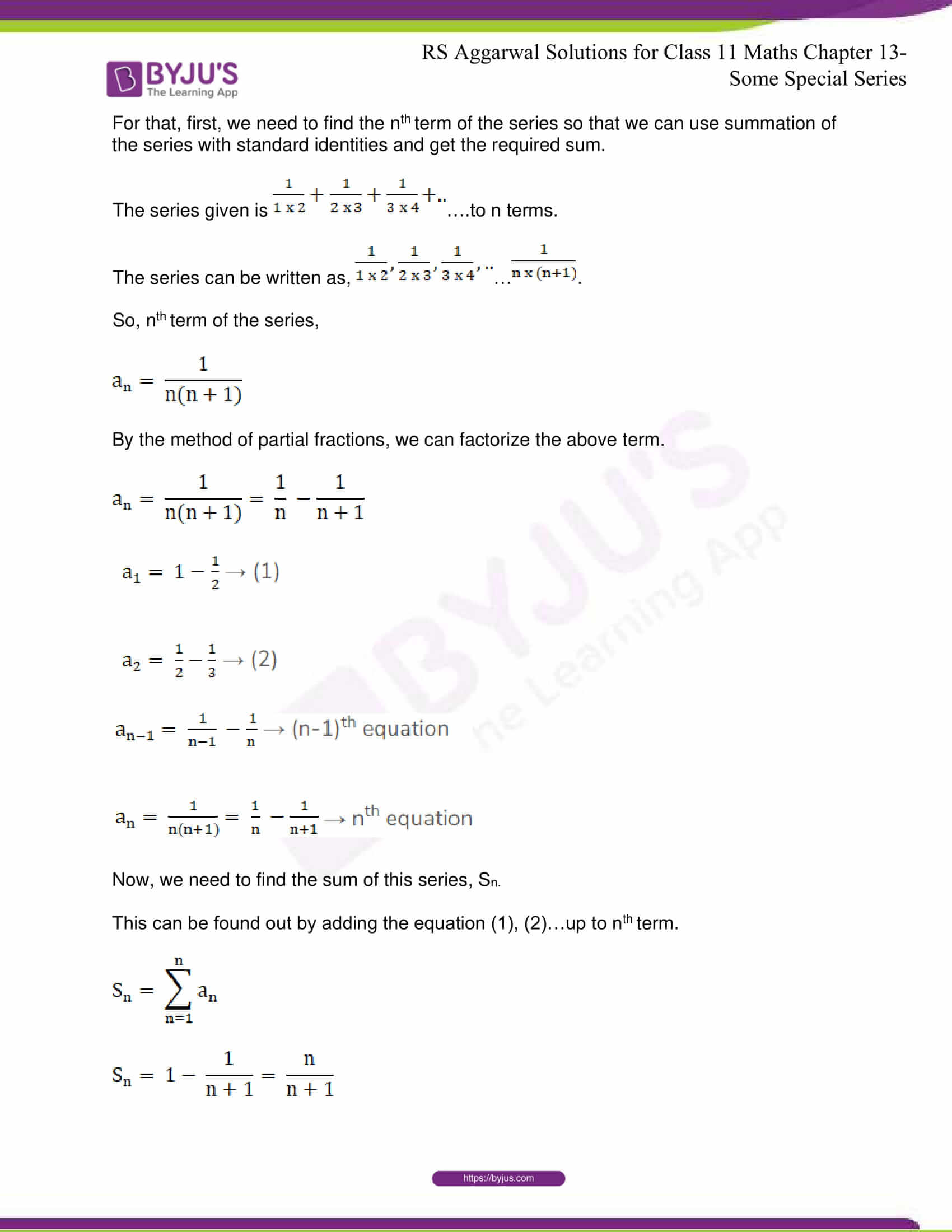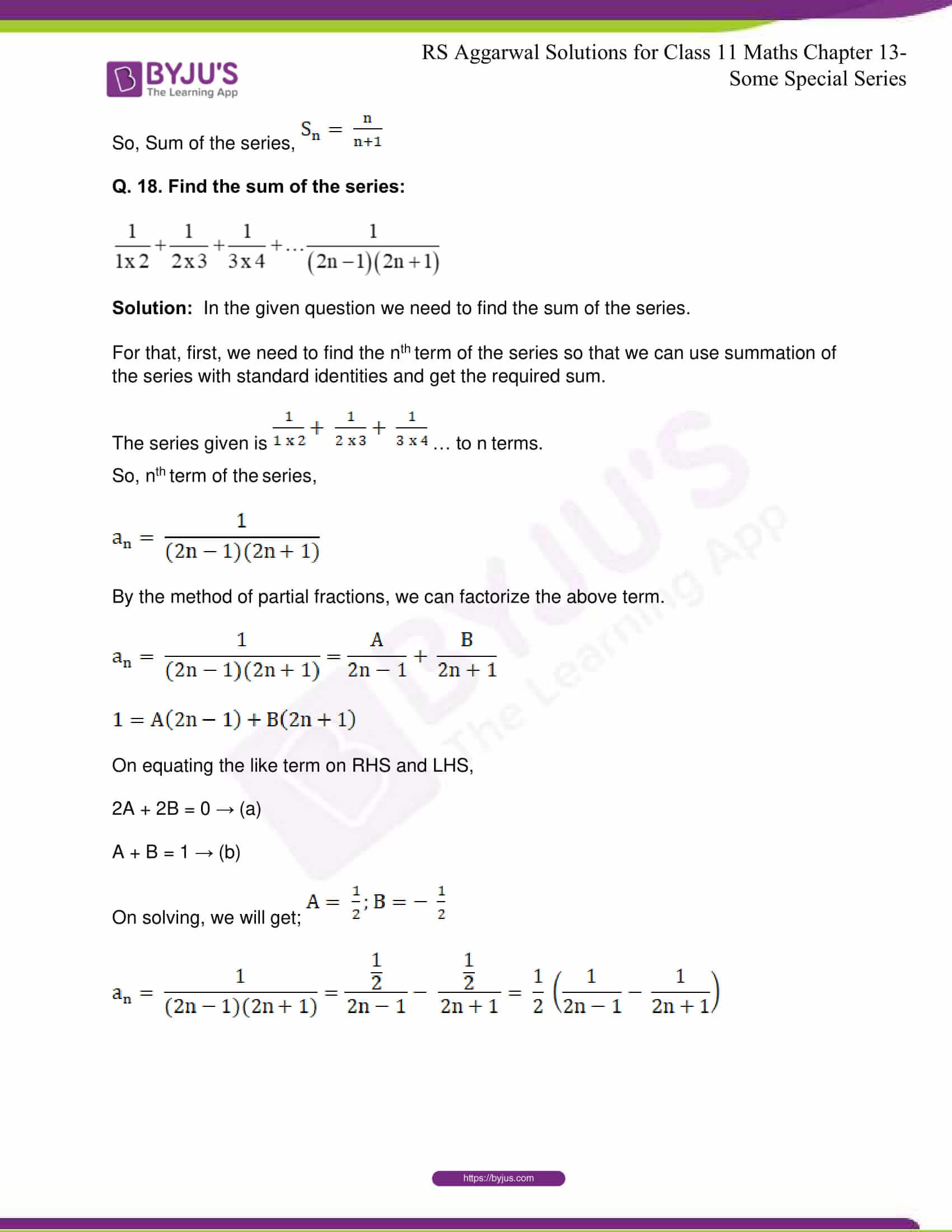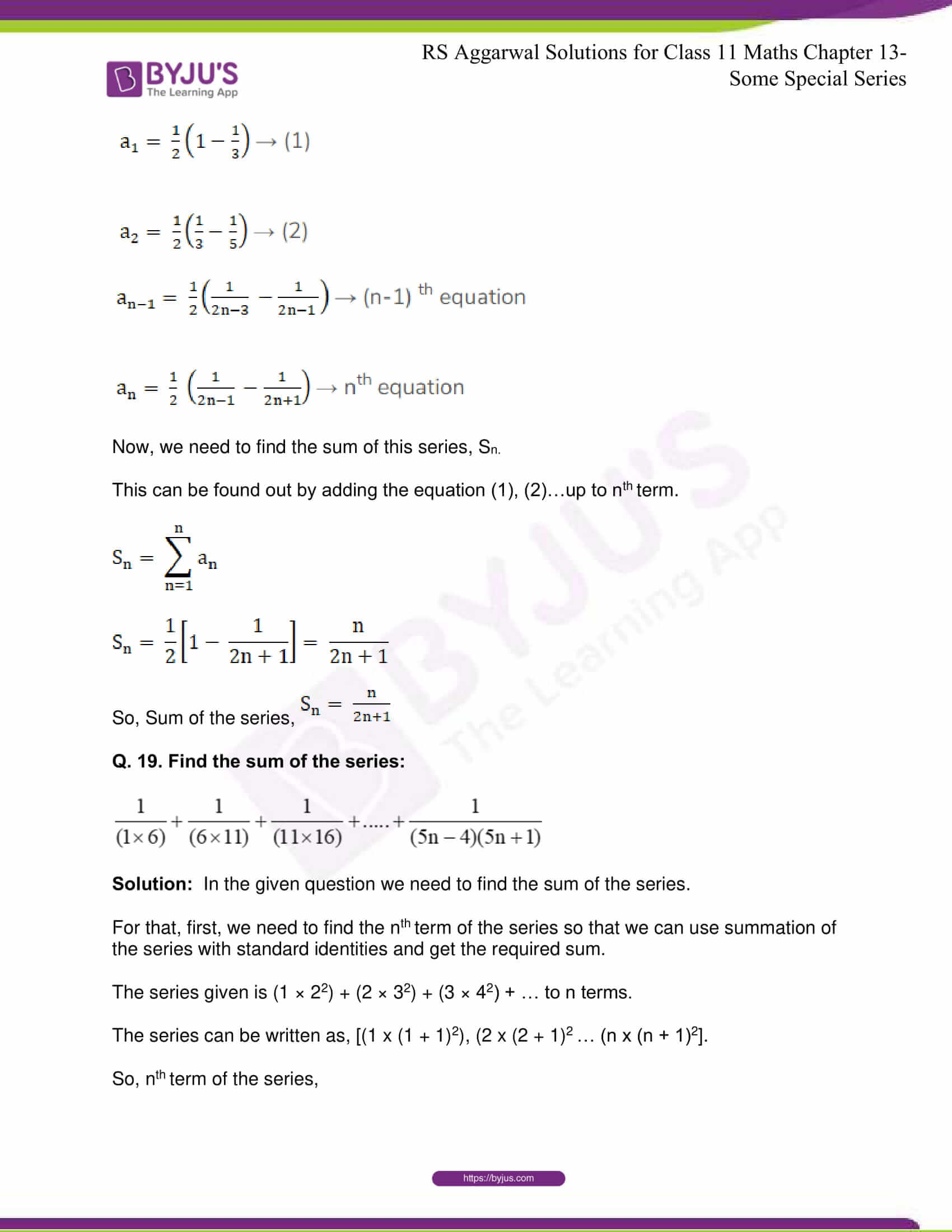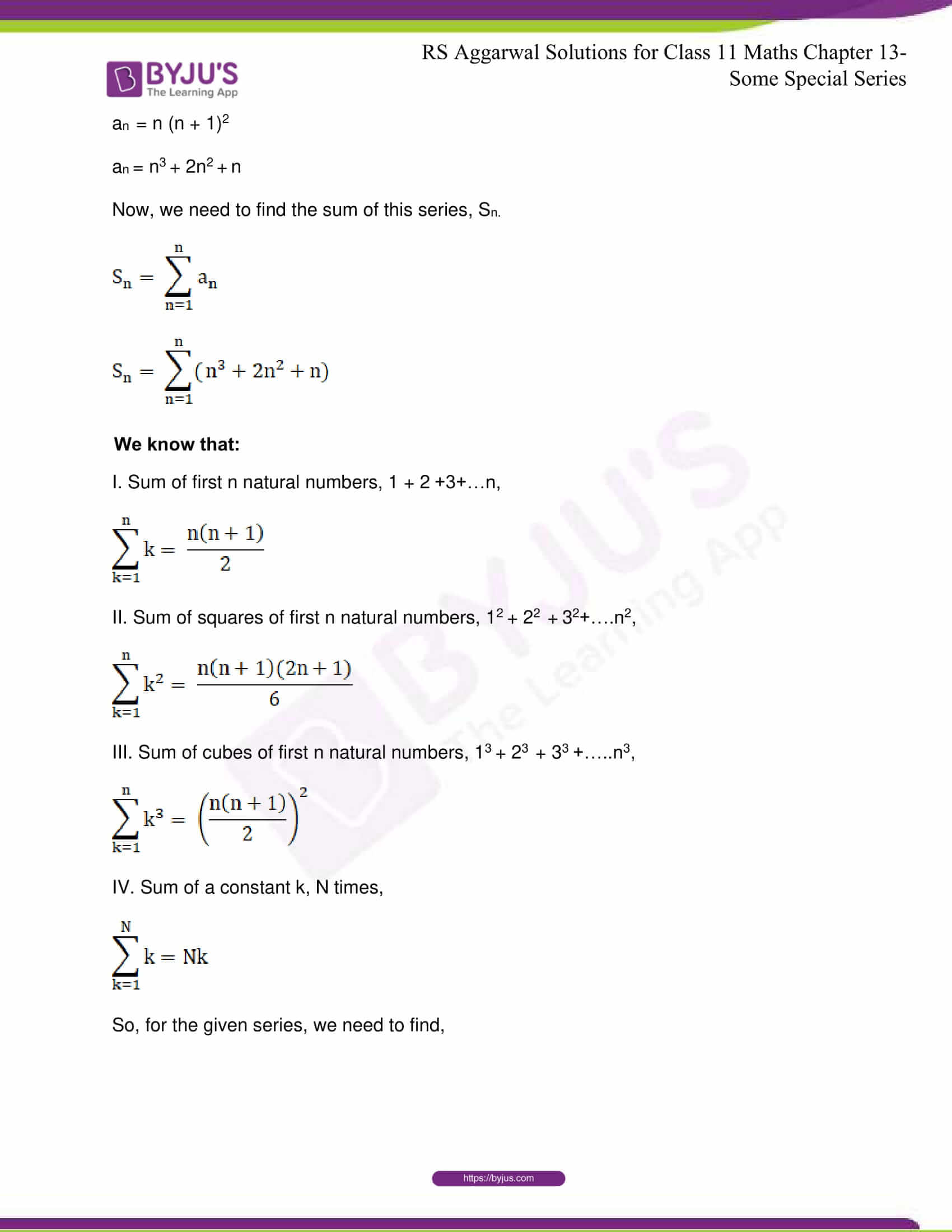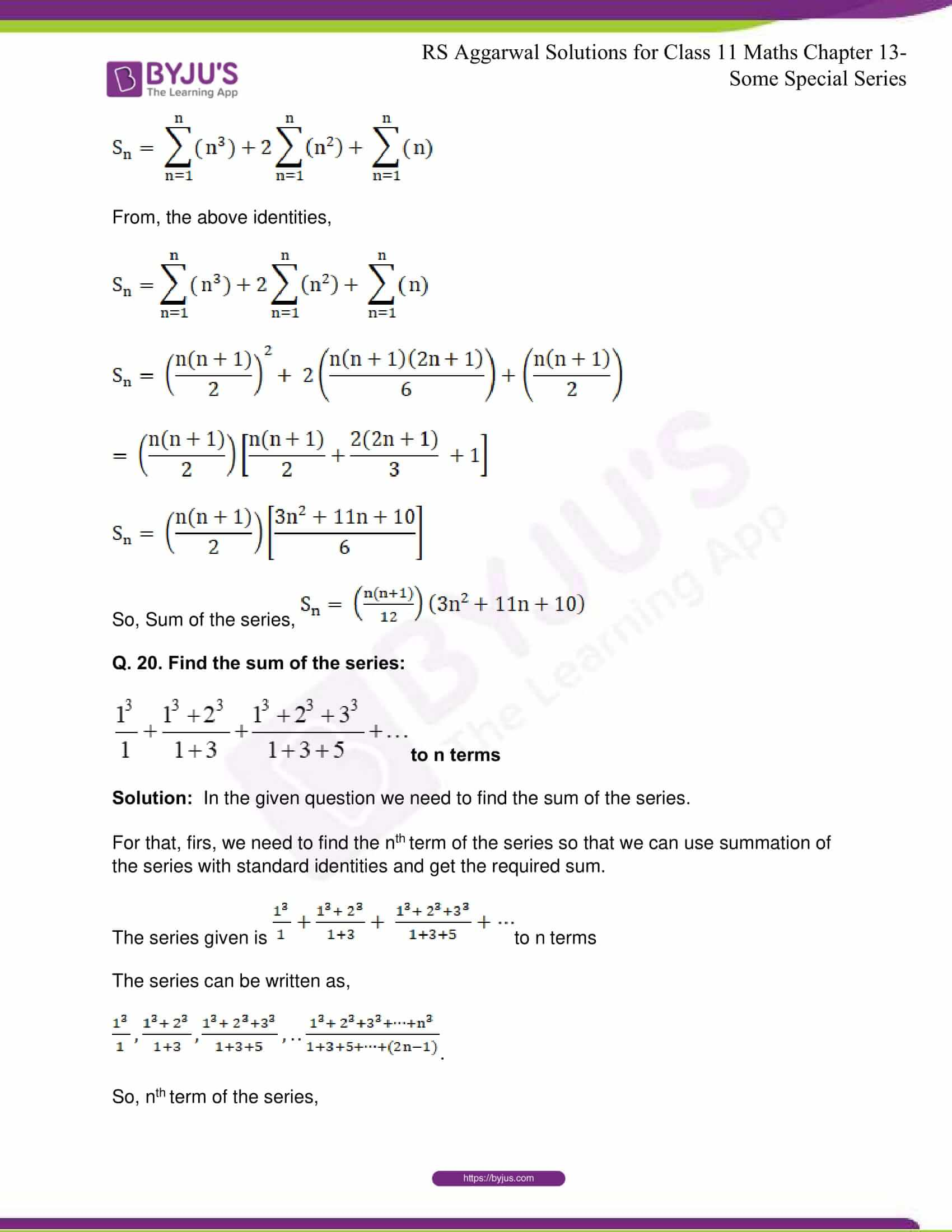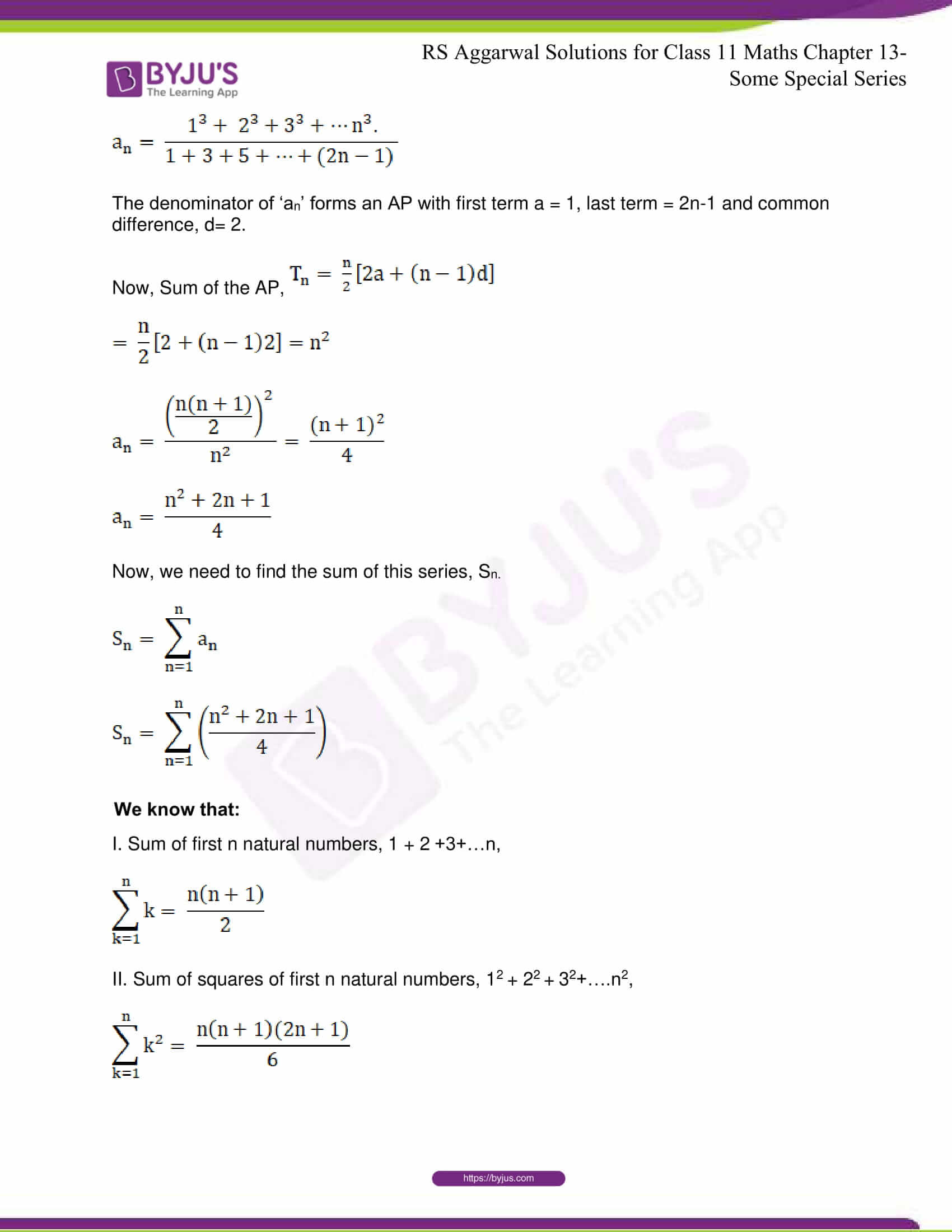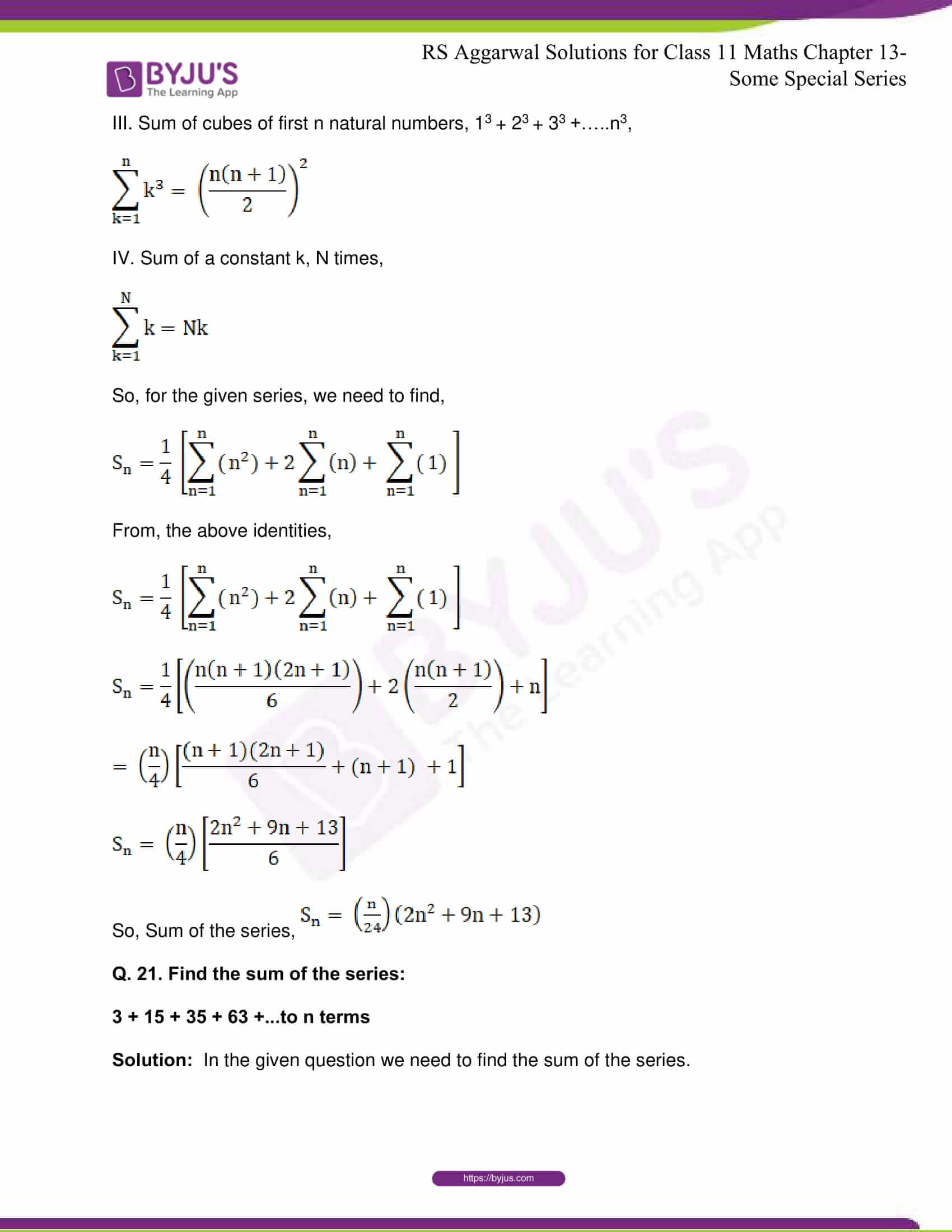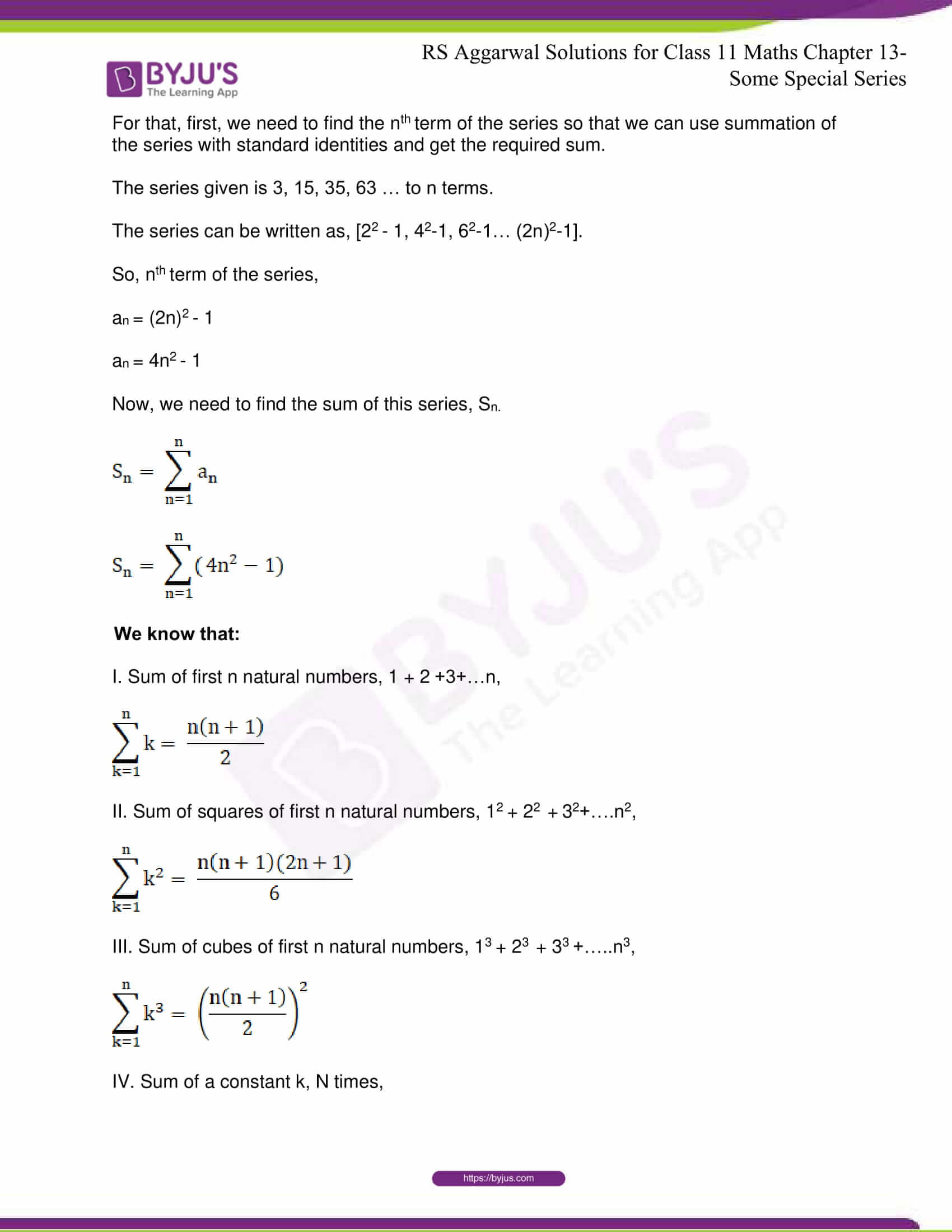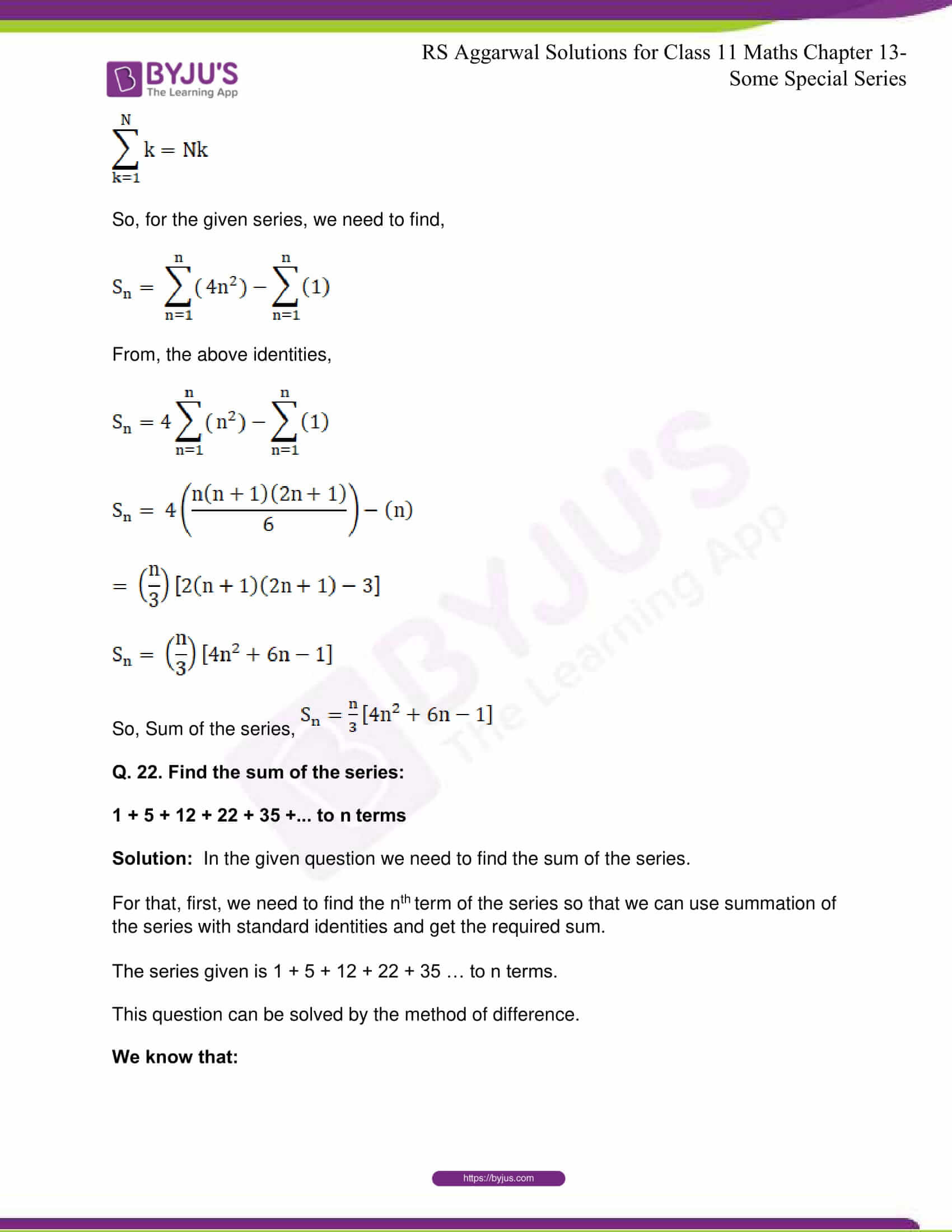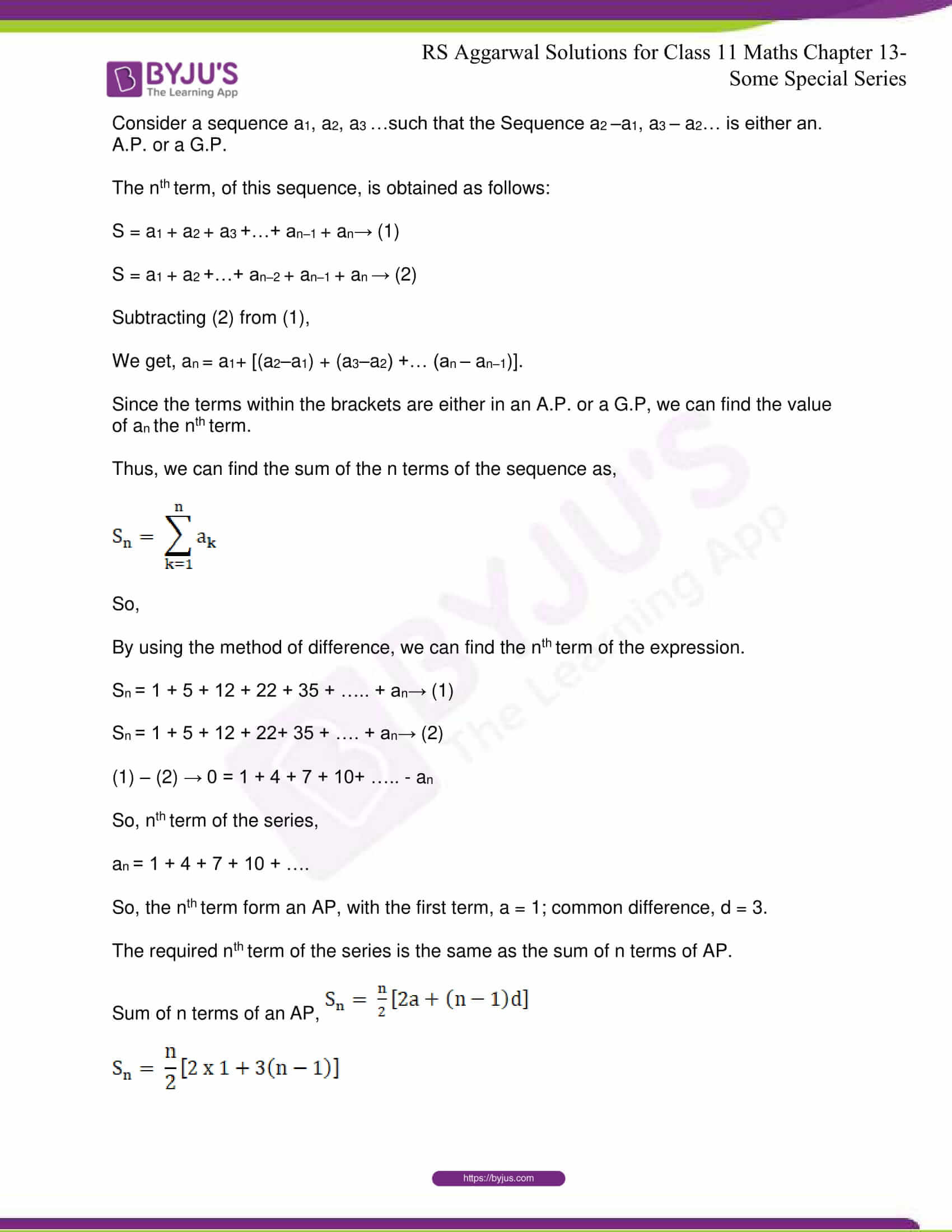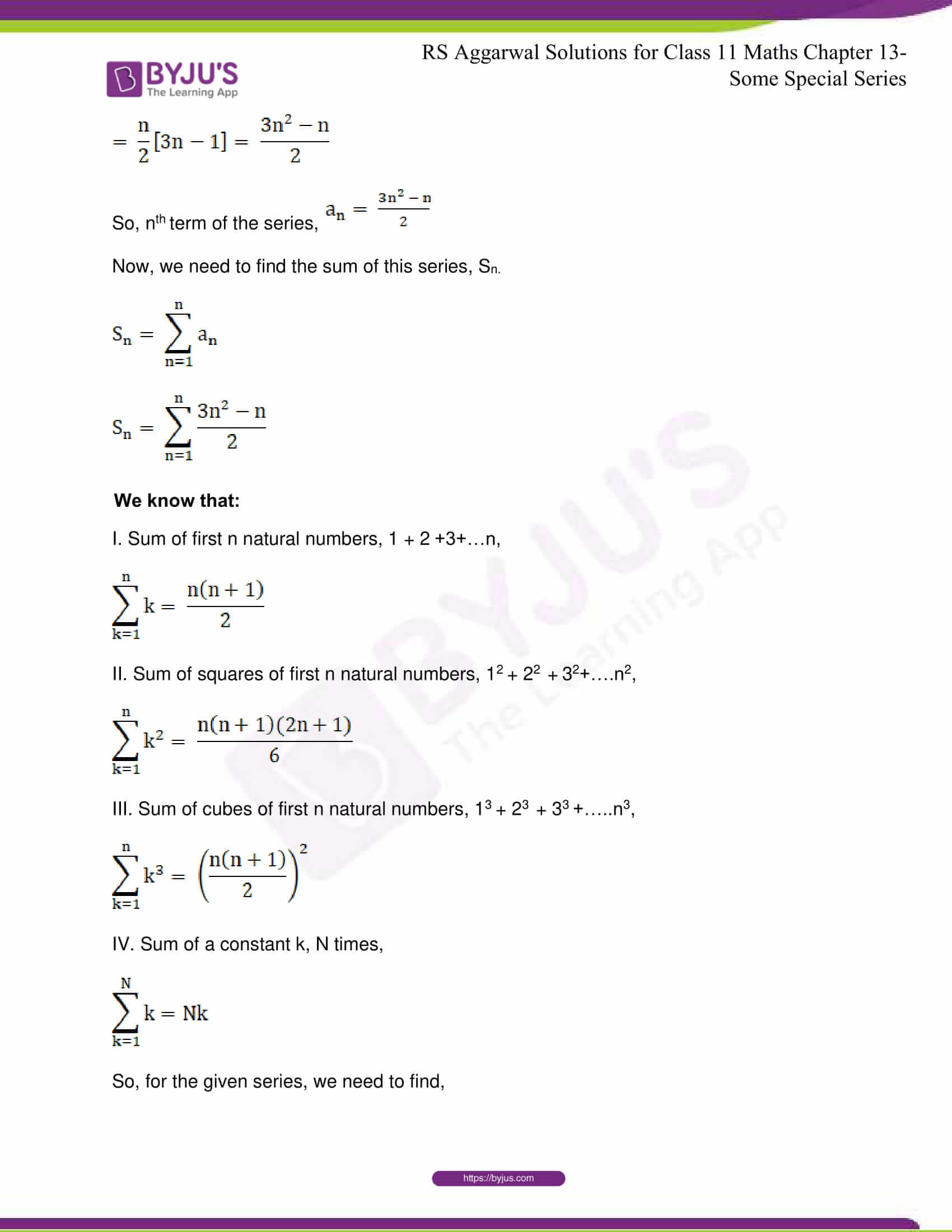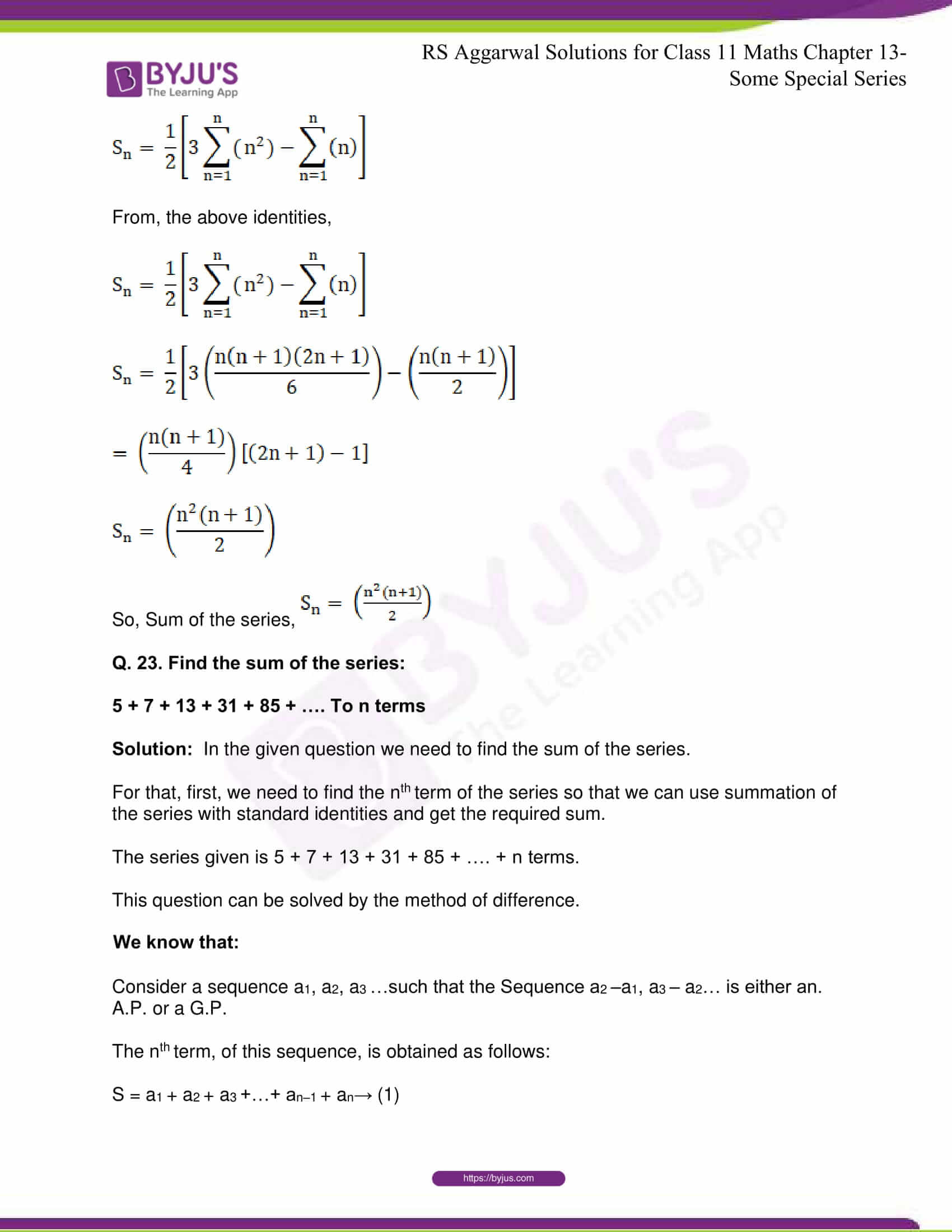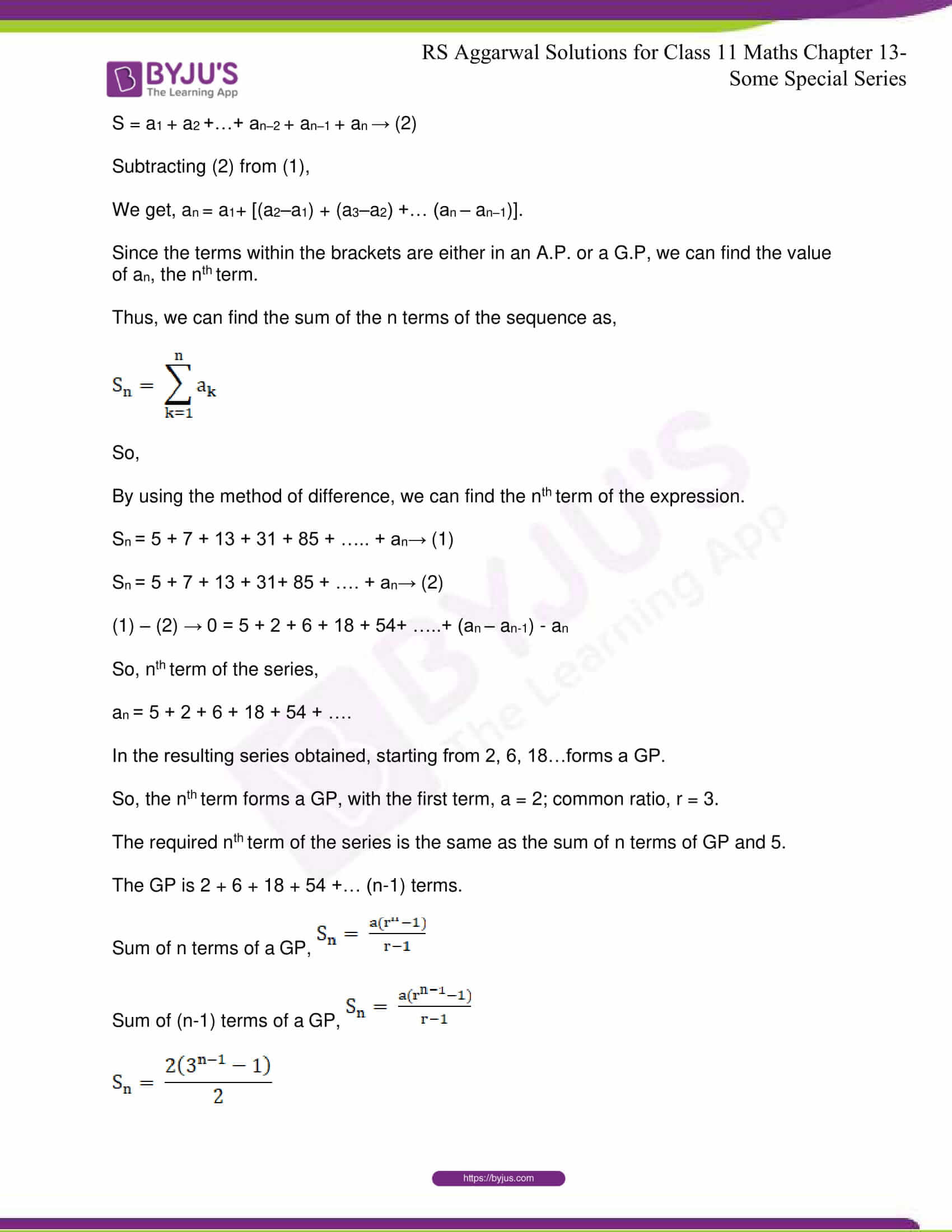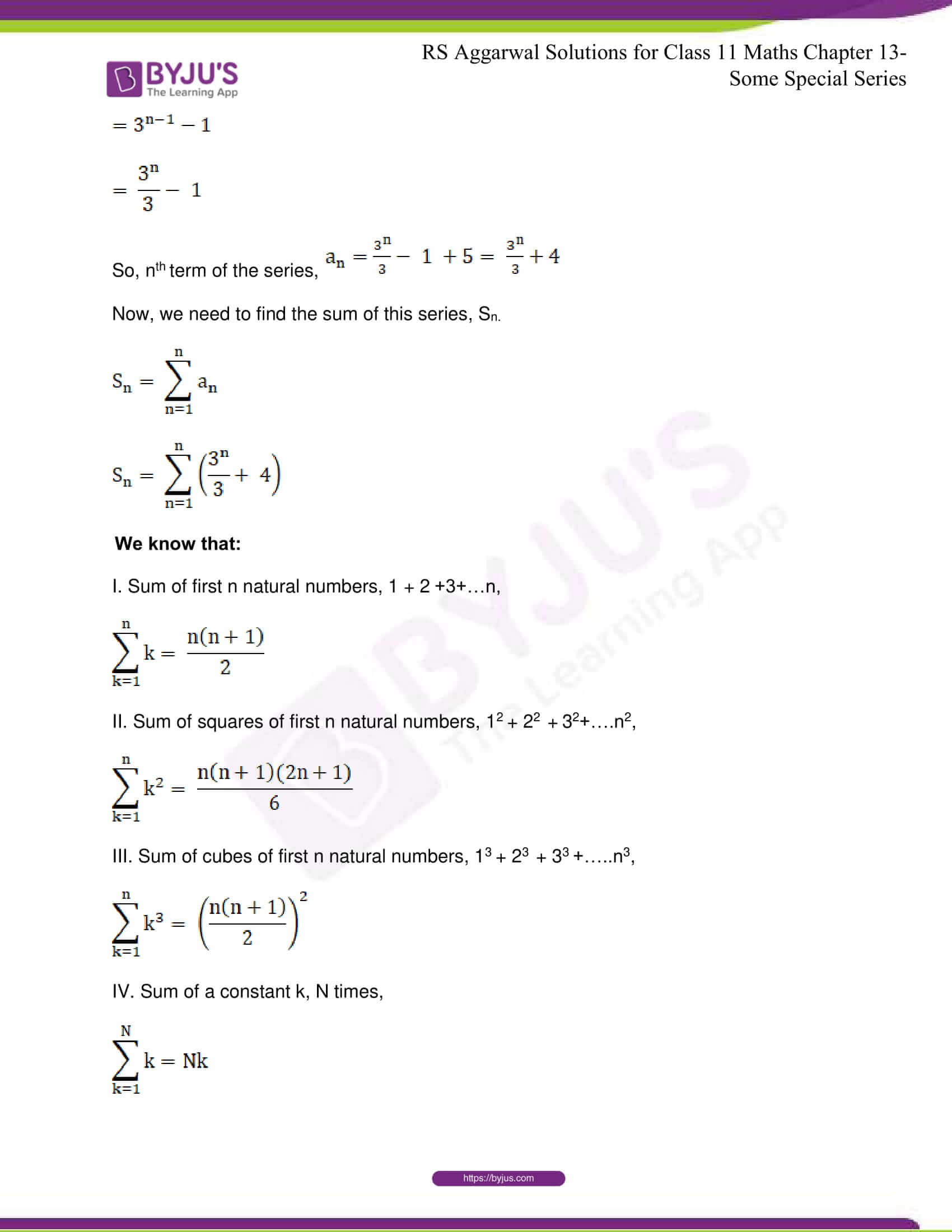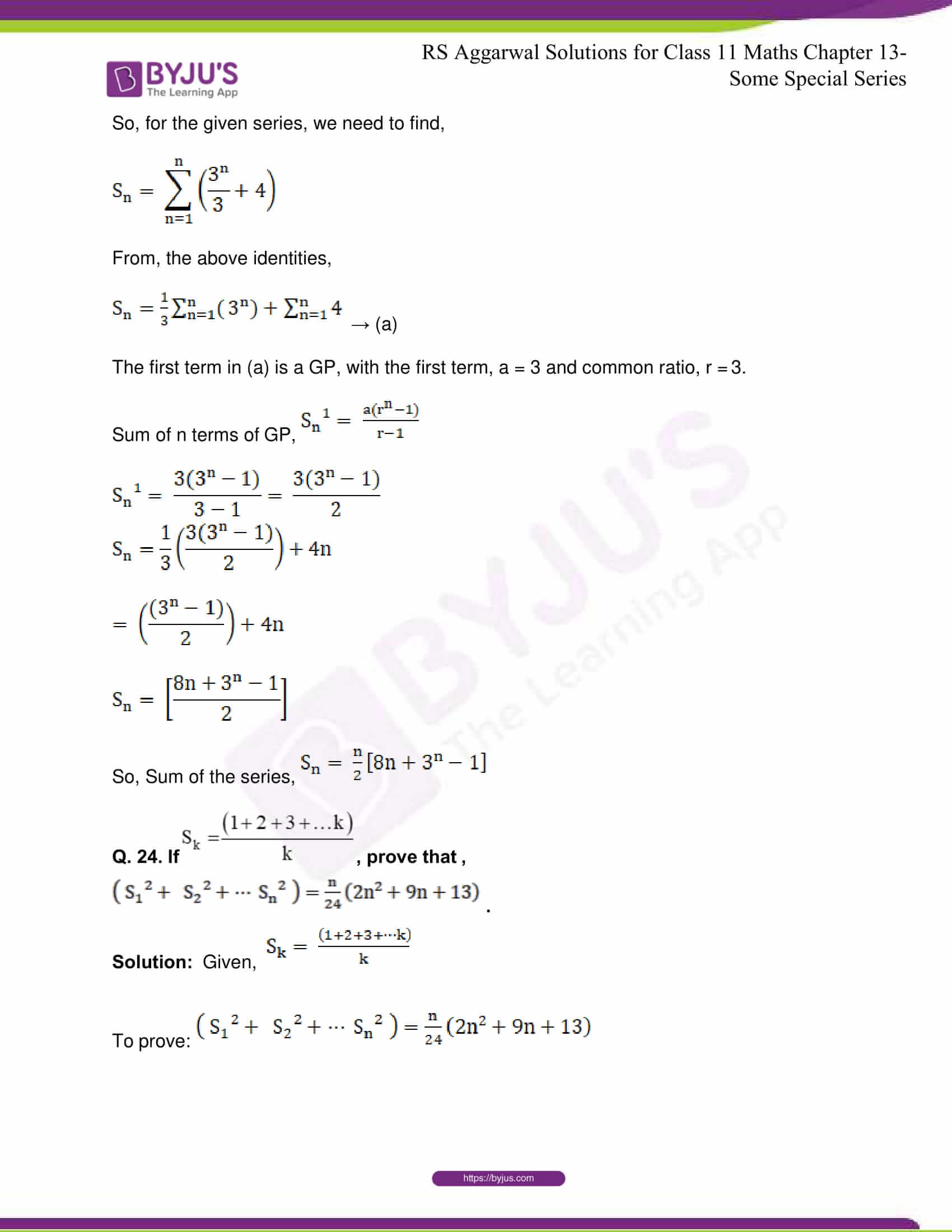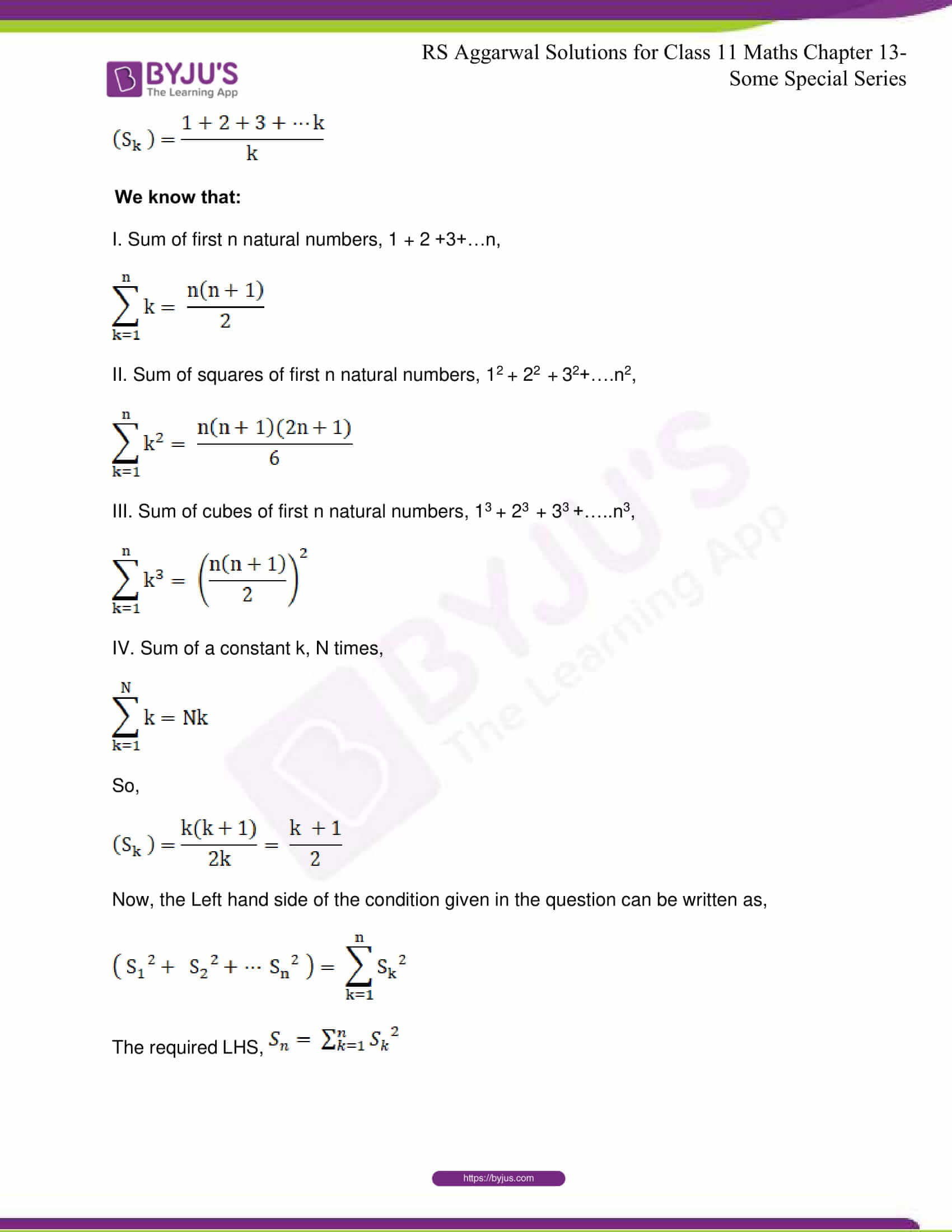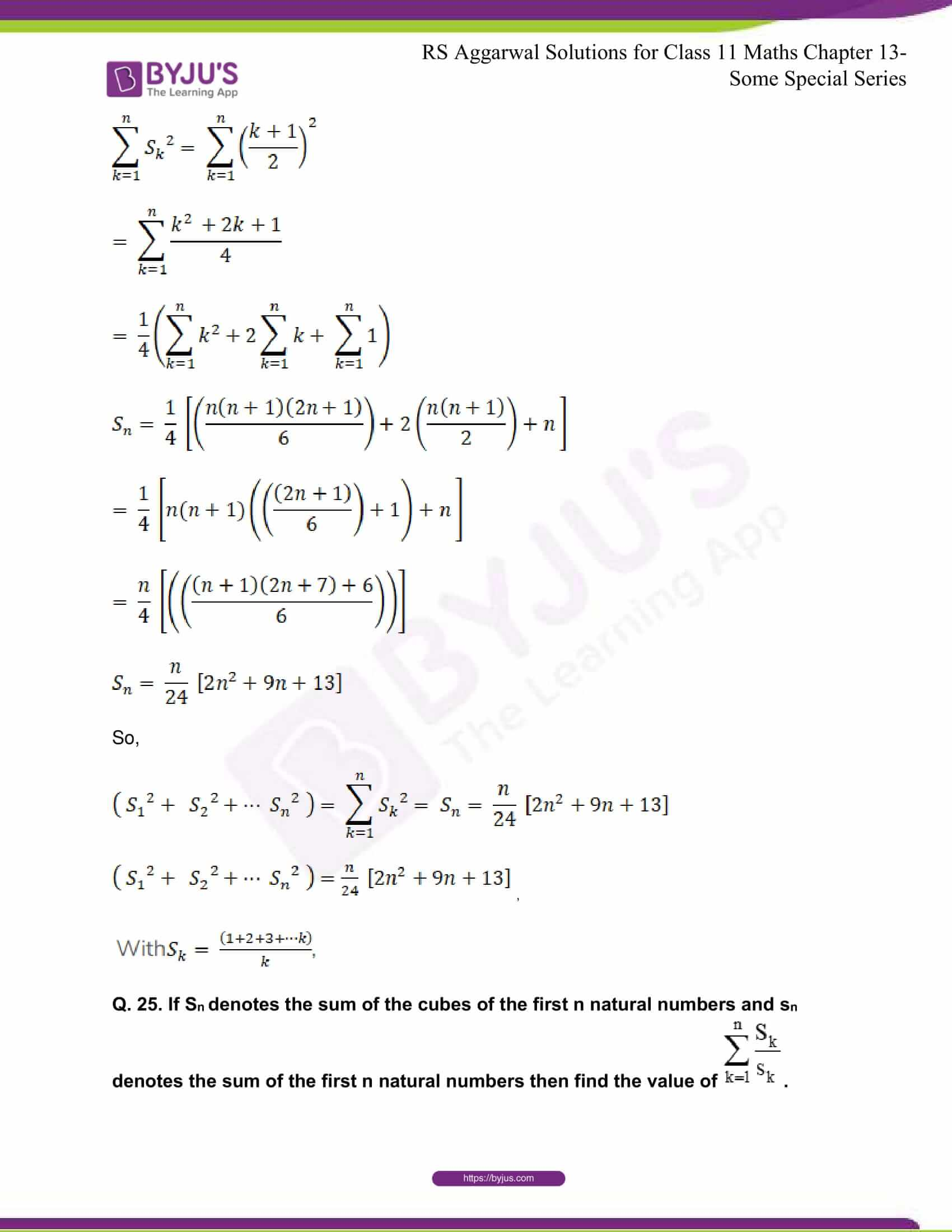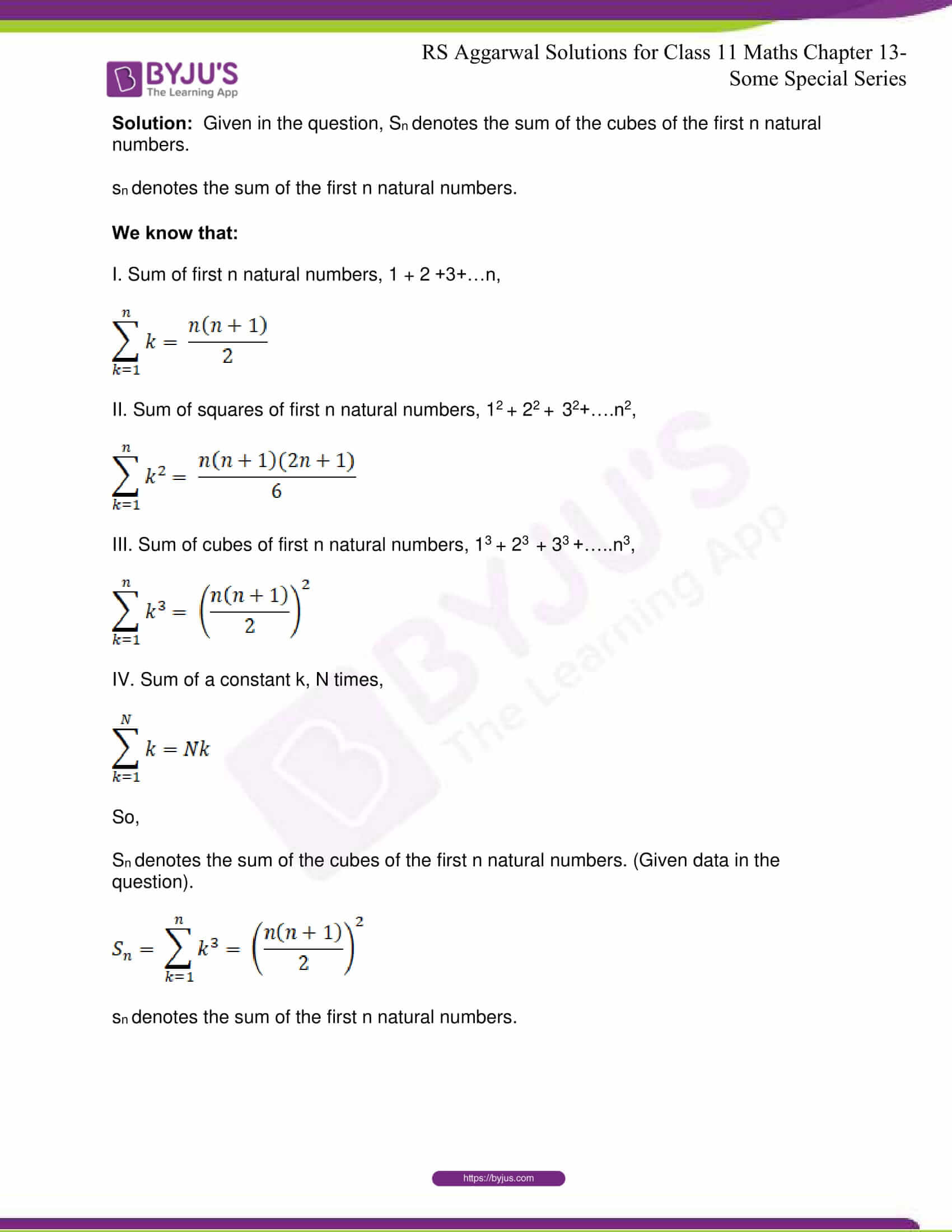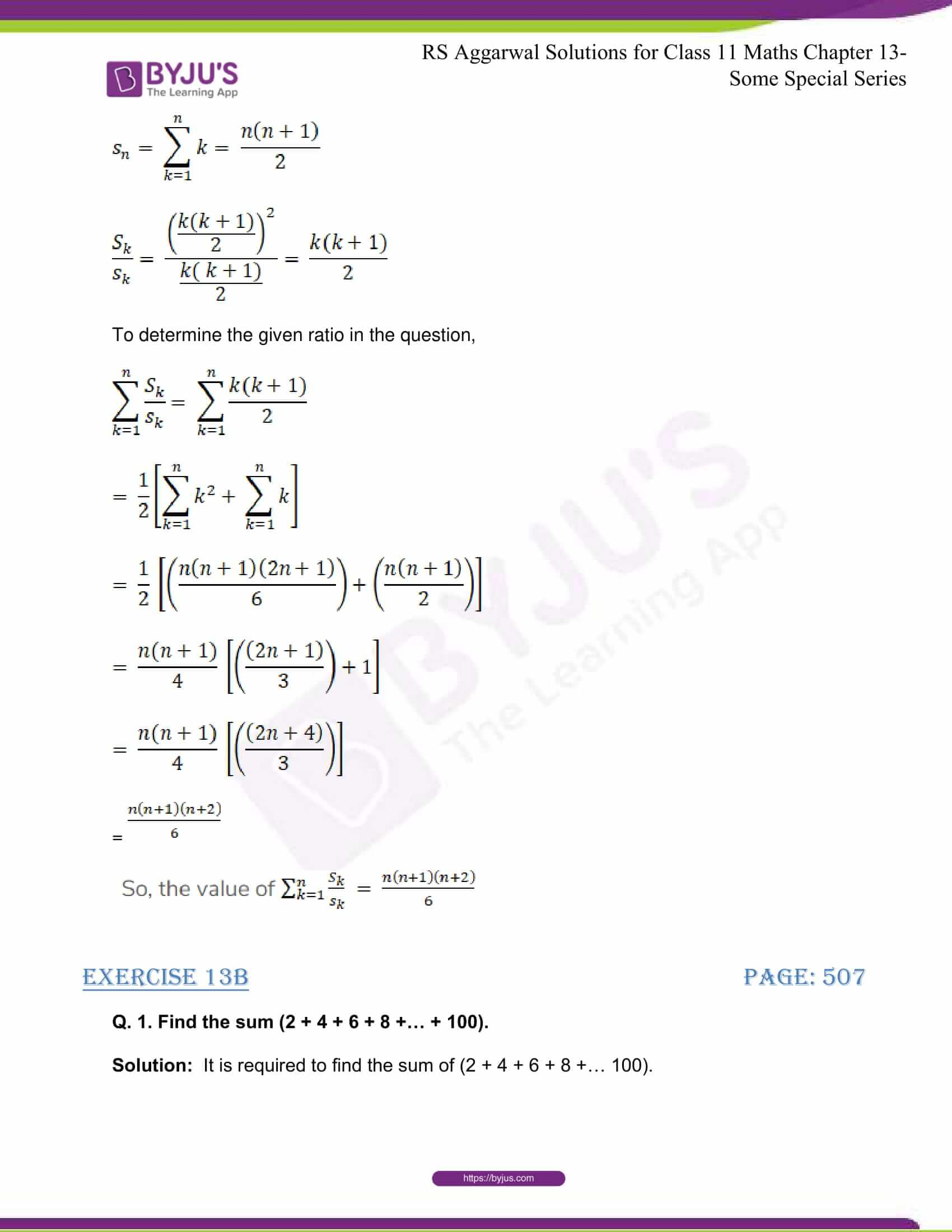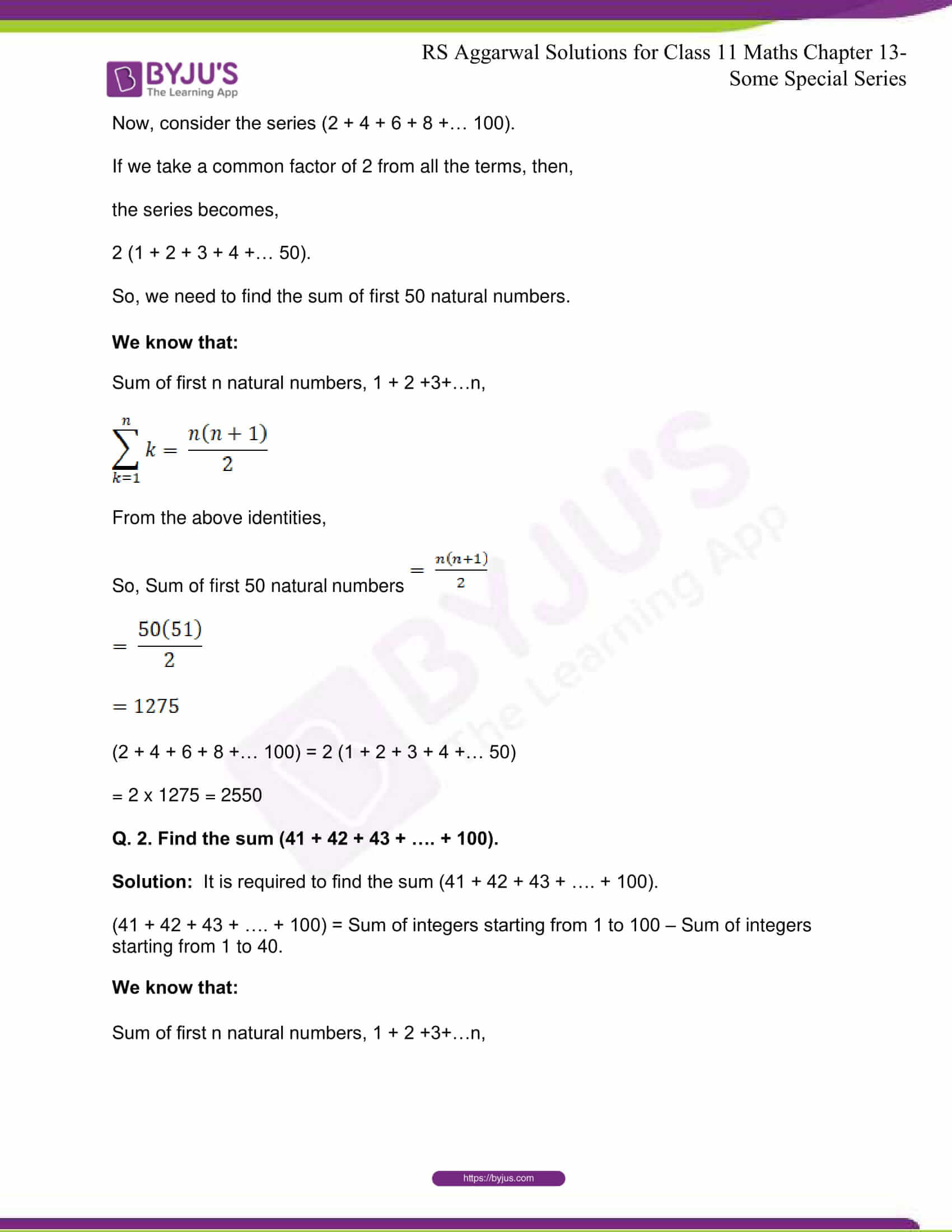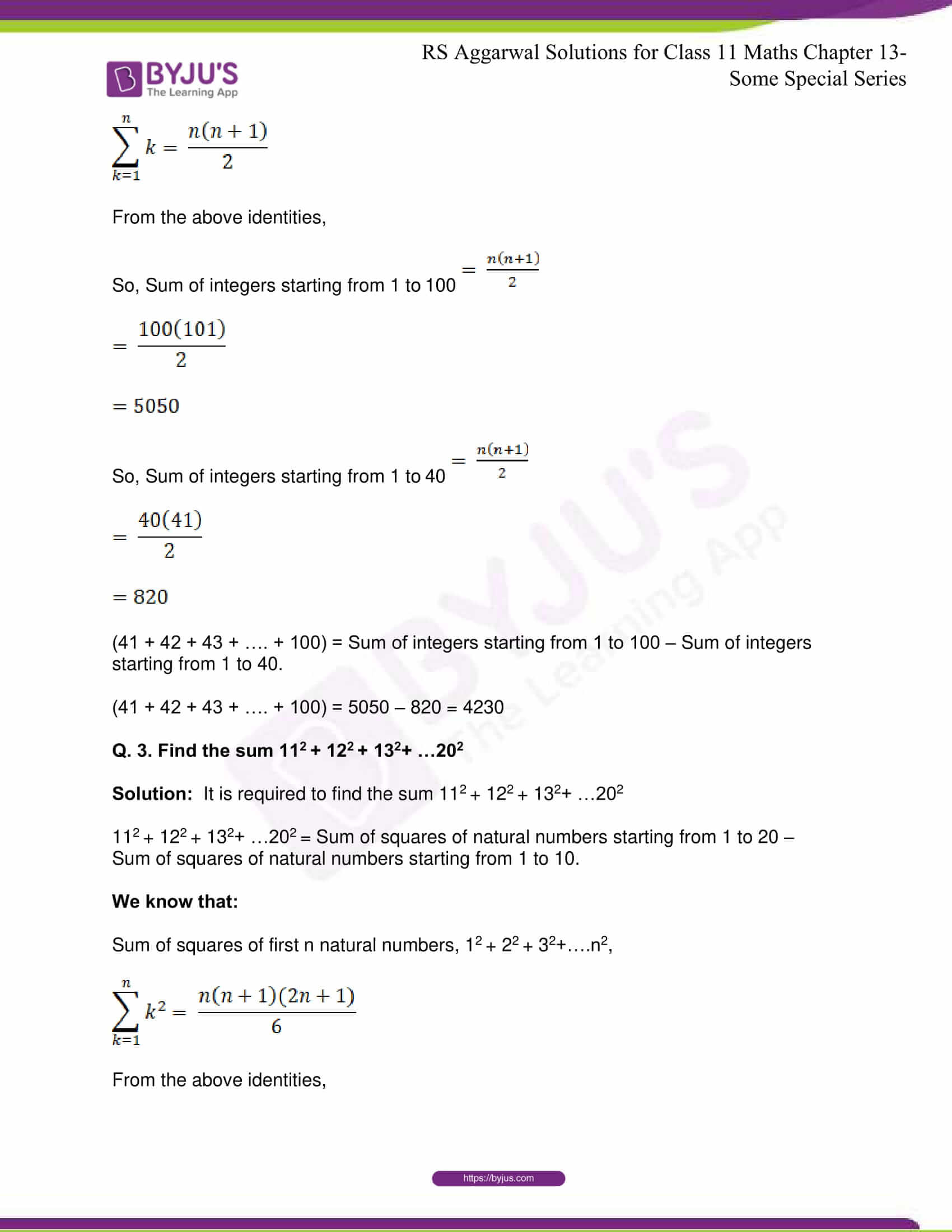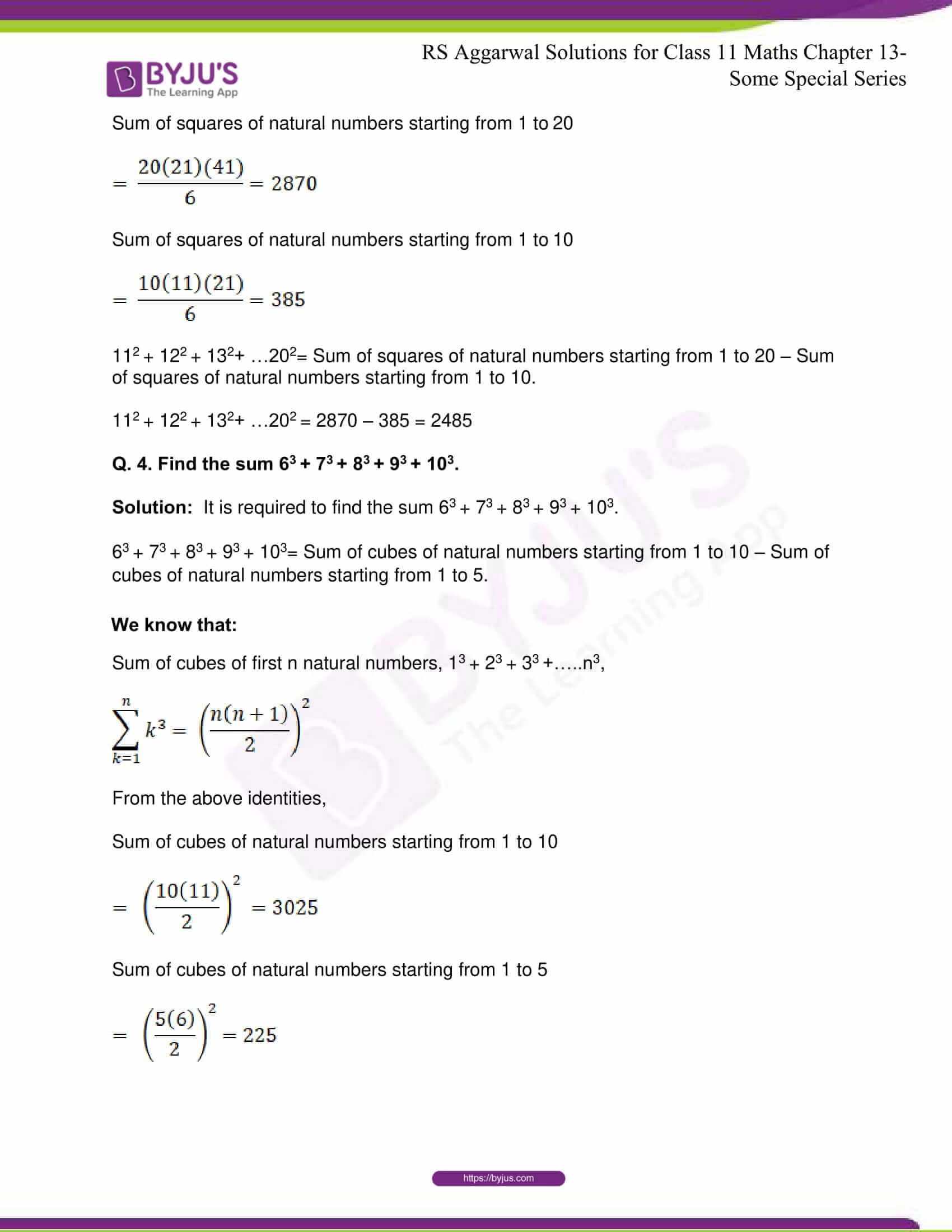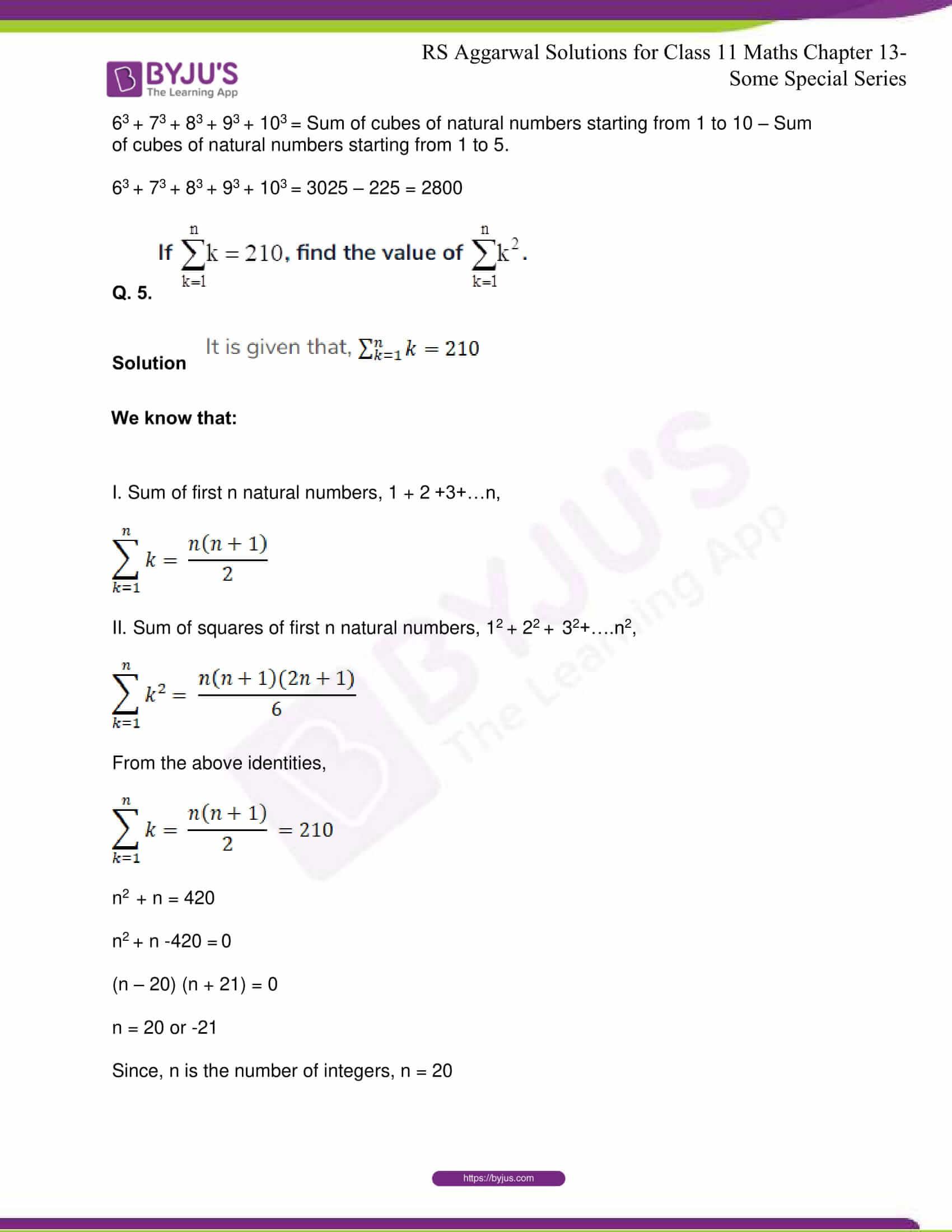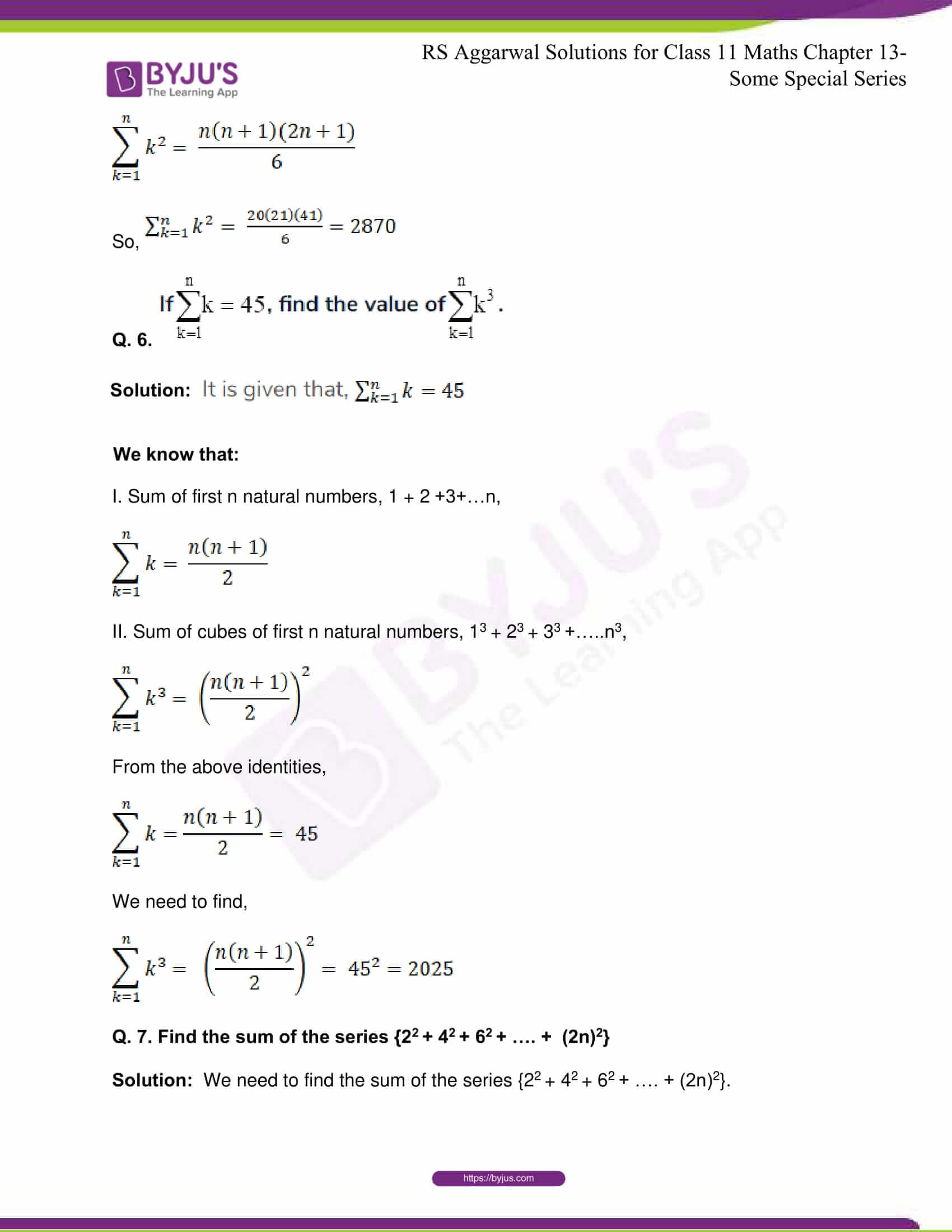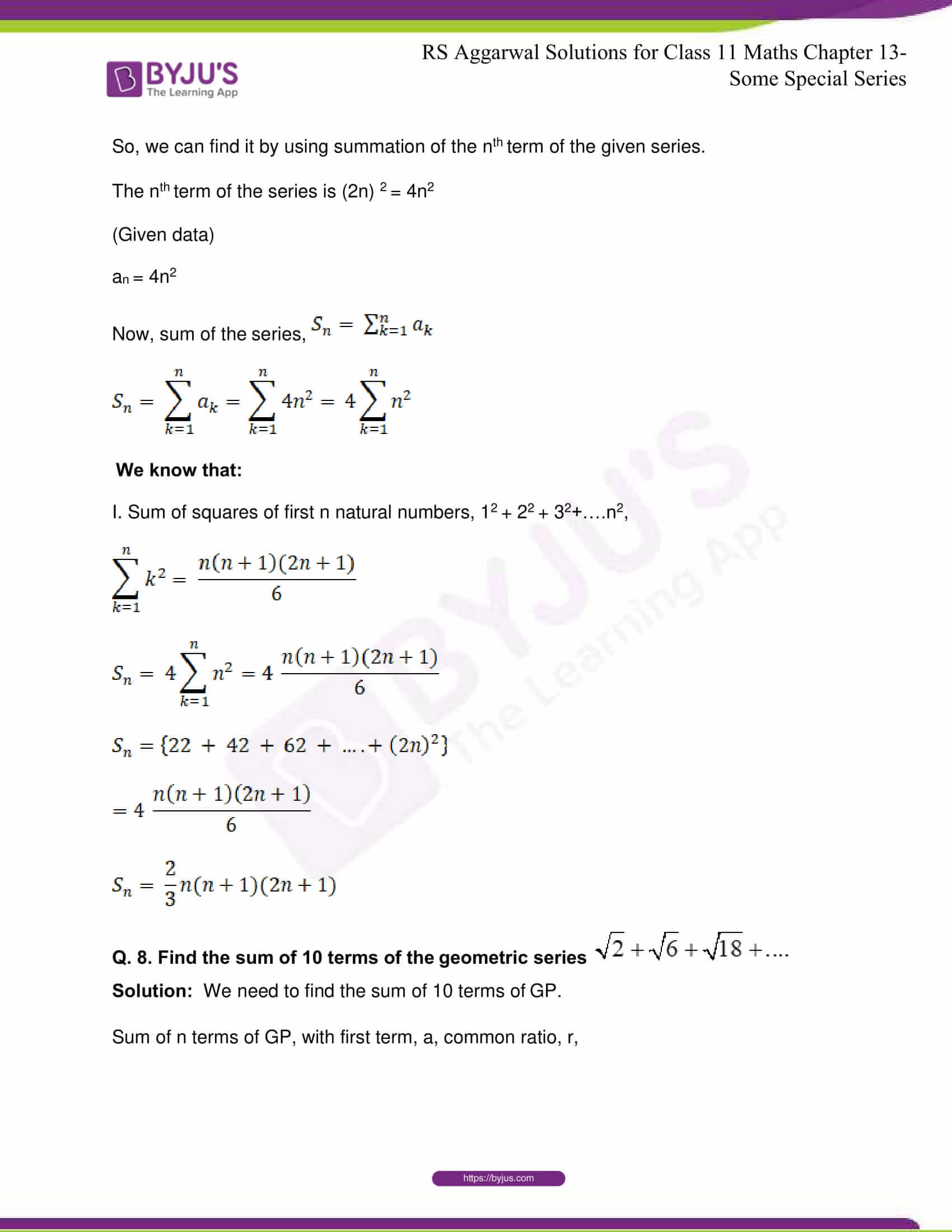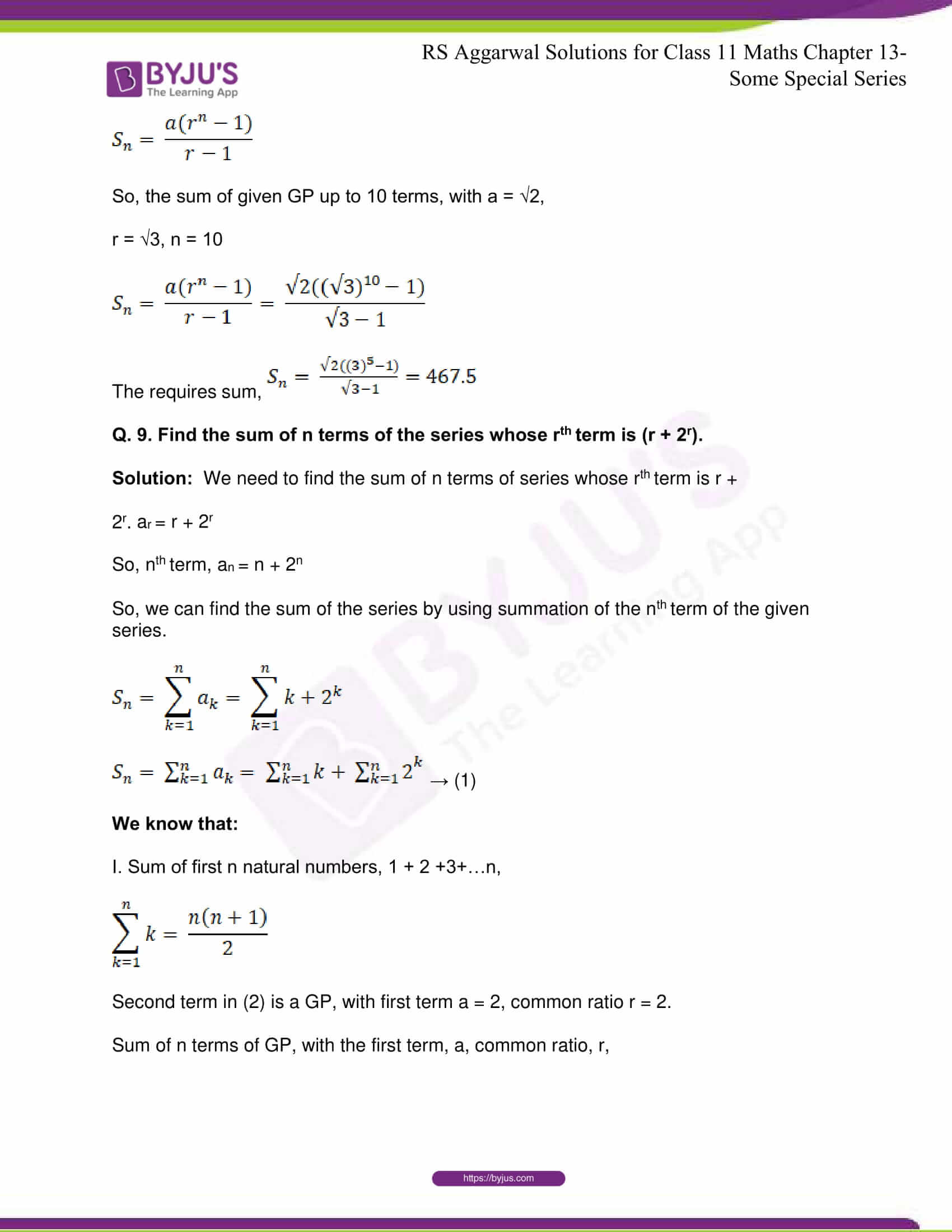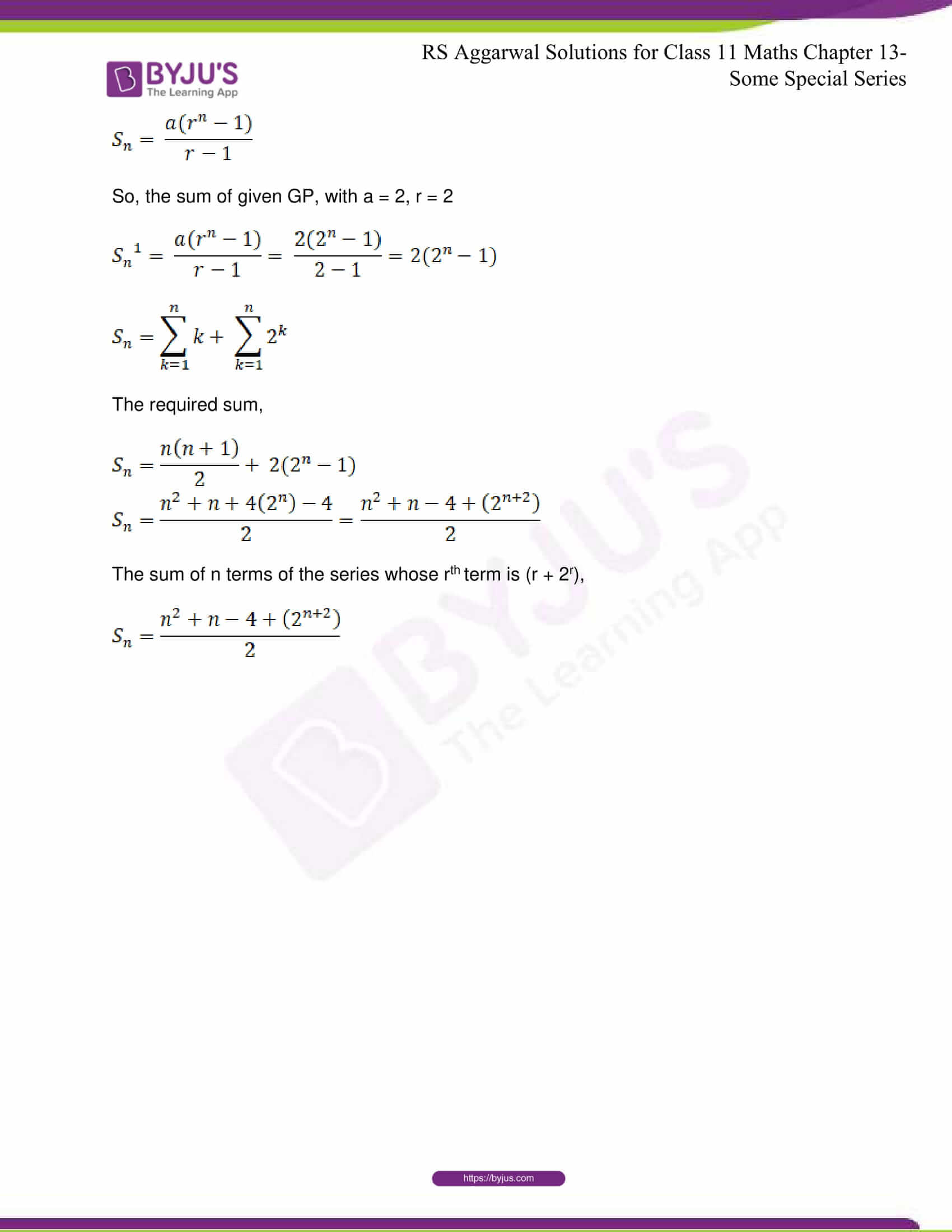## R S Aggarwal Solutions for Class 11 Maths Chapter 13- Some Special Series

The Chapter 13, Some Special Series, has 2 exercises and the R S Aggarwal Solutions given here contains the answers to all the questions present in these exercises. Let us have a look at some of the topics that are being discussed in this chapter.

13.1 Sum of first n natural numbers

13.2 Sum of the squares of first n natural numbers

13.3 Sum of the cubes of first n natural numbers

13.4 Sum of the cubes of first n odd natural numbers

## R S Aggarwal Solutions for Class 11 Maths Chapter 13-Some Special Series

There are several series present in the vast subject of Maths. Chapter 13 of class 11 teaches the students about some special series present in Mathematics. Read and learn Chapter 13 of R S Aggarwal textbook to learn more about these special series, along with the concepts covered in it. Solve the R S Aggarwal Solutions for Class 11 effectively to score high in the examination.